• 循环神经网络rnn
千次阅读
2022-04-06 20:06:30

## 一、数据介绍

本次实验采用的数据集是SNLI数据集，是 500,000 标记为英语的句子对。包括蕴含、矛盾，中立三种。
蕴含: 可以通过前提推断出假设。
矛盾: 可以推断出与假设相反。
中立: 所有其他情况。
下载数据集：

import collections
from d2l import mxnet as d2l
from mxnet import gluon, np, npx
npx.set_np()

d2l.DATA_HUB['SNLI'] = ('https://nlp.stanford.edu/projects/snli/snli_1.0.zip',
'9fcde07509c7e87ec61c640c1b2753d9041758e4')



## 二、词嵌入

使用自然语言(或由离散的单个单元组成的任何序列)的规范方法是将每个单词转换为一个单热编码的向量，并将其用于网络的下一阶段。这种方法的缺点是，随着词汇表中单词数目的增加，输入层的大小也会增加。选择的嵌入算法是GloVe，GloVe是一个基于计数的模型，在其中我们制作了一张巨大的表格，该表格显示了每个单词对应于其他单词的频数。显然，如果词汇量很高，并且使用的是诸如Wikipedia之类的大型文本数据集，那么其将形成一张巨大的表格。因此，我们对该表进行降维，以获得大小合理的词嵌入矩阵。

inputs = data.Field(lower=True)

train, dev, test = datasets.SNLI.splits(inputs, answers)

inputs.build_vocab(train, dev, test)
vector = os.path.join(USERHOME, '.vector_cache', 'glove.6B.300d.txt.pt')


## 三、RNN单元

一个RNN单元能够逐一处理句子中的所有单词。最初，将句子中的第一个单词传递给RNN单元，RNN单元生成输出和中间状态。该状态是序列的连续含义，由于在完成对整个序列的处理之前不会输出此状态，所以将其称为隐藏状态。在处理第一个单词之后，我们有了由RNN单元生成的输出和隐藏状态。输出和隐藏状态都有各自的用途。输出可以被训练以预测句子中的下一个字符或单词。这是大多数语言模型任务的工作方式。

我们将为每个单词使用相同的RNN单元，并将由上一个单词处理生成的隐藏状态作为当前单词的输入进行处理。因此，RNN单元在每个单词处理阶段都有两个输入:单词本身和上一次执行得到的隐藏状态。流程图如下：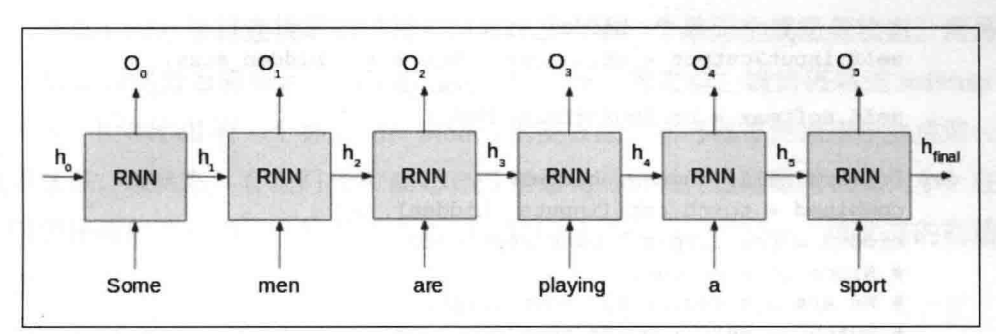实现代码如下：

class RNNCell(nn.Module):
def __init__(self, embed_dim, hidden_size, vocab_dim):
super().__init__()

self.hidden_size = hidden_size
self.input2hidden = nn.Linear(embed_dim + hidden_size, hidden_size)
# Since it's encoder
# We are not concerened about output
# self.input2output = nn.Linear(embed_dim + hidden_size, vocab_dim)
# self.softmax = nn.LogSoftmax(dim=1)

def forward(self, inputs, hidden):
combined = torch.cat((inputs, hidden), 2)
hidden = torch.relu(self.input2hidden(combined))
# Since it's encoder
# We are not concerened about output
# output = self.input2output(combined)
# output = self.softmax(output)
return hidden

def init_hidden(self, batch_size):



下面是RNN单元流程图，我们有两个全连接层，它们负责创建输出和输入的隐藏状态。RNNCell的 forward函数接受当前的输入和前一个状态的隐藏状态，然后将它们连接在一起。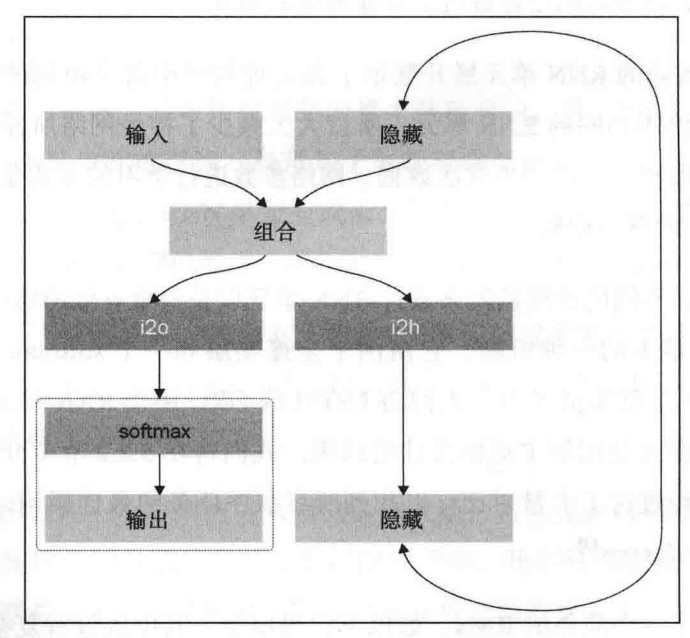一个Linear 层以连接后的张量为输入,并为下一个单元生成隐藏状态。而另一个Linear层则为当前单元生成输出。在返回训练迭代之前，输出将通过softmax进行传递。RNNCell有一个称为init_hidden 的类方法，它可以方便地用于生成第一个隐藏状态，该状态具有我们在从RNNCell初始化对象时传递的隐藏状态大小。在开始遍历序列以获取第一个隐藏状态之前，我们将调用init_hidden，该状态的初始值为零。
四、编码器

class Encoder(nn.Module):

def __init__(self, embed_dim, vocab_dim, hidden_size):
super(Encoder, self).__init__()
self.rnn = RNNCell(embed_dim, hidden_size, vocab_dim)

def forward(self, inputs):
# .size(1) dimension is batch size
ht = self.rnn.init_hidden(inputs.size(1))
for word in inputs.split(1, dim=0):
ht = self.rnn(word, ht)
return ht


在forward函数中，首先将RNNCell的隐藏状态初始化为零，这可以通过调用之前创建的 init_hidden方法来完成。然后将输入序列在第一维上按大小为1进行拆分，然后进行遍历。这是在假设输入为batch_first之后进行的，因此第一维将是序列长度。为了遍历每个单词，必须遍历第一维。

对于每个单词，使用当前单词(输入）和前一个状态的隐藏状态来调用self.rnn的forward 函数。self.rnn返回下一个单元的输出和隐藏状态，继续循环直到序列结束。对于问题场景，不必担心输出，也不对从输出中获得的损失进行反向传播。相反，假设最后一个隐藏状态拥有句子的含义。

## 五、分类器

我们的网络的最后一个组件是分类器。因此，现在我们通过编码器处理了两个句子，并得到了它们的最终隐藏状态。现在是时候定义损失函数了。一种方法是计算两个句子中的高维隐藏状态之间的距离。损失可以按以下方式定义:
1）如果句子对是蕴含关系，则将损失最大化为一个较大的正值。
2）如果句子对是矛盾关系，则将损失最小化为一个较大的负值。
3)如果句子对是中性的，则将损失保持在零附近(在两个或三个边界中即可另一种方法是将两个句子的隐藏状态拼接起来，然后将它们传递到另一层集，并定义最终的分类器层，该层可以将拼接的值分类为我们想要的三个类之一。实际的SPINN实现使用该方法，但是其使用的融合机制比简单的拼接更为复杂。
代码如下：

class Merger(nn.Module):

def __init__(self, size, dropout=0.5):
super().__init__()
self.bn = nn.BatchNorm1d(size)
self.dropout = nn.Dropout(p=dropout)

def forward(self, data):
prem = data
hypo = data
diff = prem - hypo
prod = prem * hypo
cated_data = torch.cat([prem, hypo, diff, prod], 2)
cated_data = cated_data.squeeze()
return self.dropout(self.bn(cated_data))


在这里，Merger节点的构建是为了模拟SPINN的实际实现。Merger的forward函数入参为两个序列: prem 和hypoo。我们是首先通过减法确定两个句子间的差异，然后将实际句子与其间的差异和计算得到的乘积连接起来，并将其传递给批次归一化层和 dropout层。
下面将所有组件封装起来

class RNNClassifier(nn.Module):

def __init__(self, config):
super().__init__()
self.embed = nn.Embedding(config.vocab_dim, config.embed_dim)
self.encoder = Encoder(
config.embed_dim, config.vocab_dim, config.hidden_size)
self.classifier = nn.Sequential(
Merger(4 * config.hidden_size, config.dropout),
nn.Linear(4 * config.hidden_size, config.fc1_dim),
nn.ReLU(),
nn.BatchNorm1d(config.fc1_dim),
nn.Dropout(p=config.dropout),
nn.Linear(config.fc1_dim, config.fc2_dim)
)

def forward(self, batch):
# breakpoint()
prem_embed = self.embed(batch.premise)
hypo_embed = self.embed(batch.hypothesis)
premise = self.encoder(prem_embed)
hypothesis = self.encoder(hypo_embed)
scores = self.classifier((premise, hypothesis))
return scores


最终序列层从 Merger节点开始。合并后的输出的序列长度维数将增加四倍,因为我们将两个句子、两个句子的差和乘积都追加到了Merger的输出中。之后该输出经过一个全连接层，然后在ReLU非线性变换后使用batchnorm1d对其进行归一化。之后的dropout降低了过拟合的概率，随后经过另一个全连接层，该层为我们的输入数据创建了得分。输入数据将决定数据所属的类别(蕴含、矛盾或中性）

## 六、dropout

dropout它消除了对通常的正则化技术的需求，而正则化技术在dropout之前一直很普遍。借助于dropout，我们随机丢弃了网络中神经元之间的连接(如下图所示)，因此网络不得不泛化并且不能偏向任何类型的外部因素。要删除神经元，只需将其输出设置为零即可。丢弃随机神经元可防止网络共适，因此在很大程度上降低了过拟合。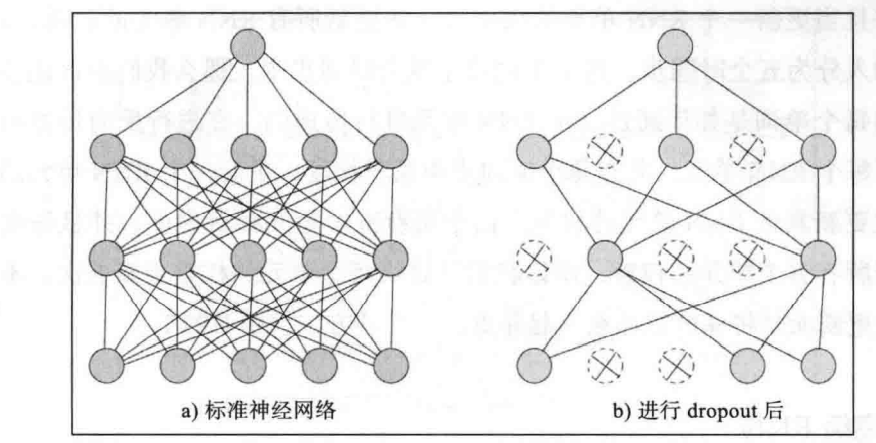完整代码如下：
model.py

import torch.nn as nn
import torch

class RNNCell(nn.Module):
def __init__(self, embed_dim, hidden_size, vocab_dim):
super().__init__()

self.hidden_size = hidden_size
self.input2hidden = nn.Linear(embed_dim + hidden_size, hidden_size)
# Since it's encoder
# We are not concerened about output
# self.input2output = nn.Linear(embed_dim + hidden_size, vocab_dim)
# self.softmax = nn.LogSoftmax(dim=1)

def forward(self, inputs, hidden):
combined = torch.cat((inputs, hidden), 2)
hidden = torch.relu(self.input2hidden(combined))
# Since it's encoder
# We are not concerened about output
# output = self.input2output(combined)
# output = self.softmax(output)
return hidden

def init_hidden(self, batch_size):

class Encoder(nn.Module):

def __init__(self, embed_dim, vocab_dim, hidden_size):
super(Encoder, self).__init__()
self.rnn = RNNCell(embed_dim, hidden_size, vocab_dim)

def forward(self, inputs):
# .size(1) dimension is batch size
ht = self.rnn.init_hidden(inputs.size(1))
for word in inputs.split(1, dim=0):
ht = self.rnn(word, ht)
return ht

class Merger(nn.Module):

def __init__(self, size, dropout=0.5):
super().__init__()
self.bn = nn.BatchNorm1d(size)
self.dropout = nn.Dropout(p=dropout)

def forward(self, data):
prem = data
hypo = data
diff = prem - hypo
prod = prem * hypo
cated_data = torch.cat([prem, hypo, diff, prod], 2)
cated_data = cated_data.squeeze()
return self.dropout(self.bn(cated_data))

class RNNClassifier(nn.Module):

def __init__(self, config):
super().__init__()
self.embed = nn.Embedding(config.vocab_dim, config.embed_dim)
self.encoder = Encoder(
config.embed_dim, config.vocab_dim, config.hidden_size)
self.classifier = nn.Sequential(
Merger(4 * config.hidden_size, config.dropout),
nn.Linear(4 * config.hidden_size, config.fc1_dim),
nn.ReLU(),
nn.BatchNorm1d(config.fc1_dim),
nn.Dropout(p=config.dropout),
nn.Linear(config.fc1_dim, config.fc2_dim)
)

def forward(self, batch):
# breakpoint()
prem_embed = self.embed(batch.premise)
hypo_embed = self.embed(batch.hypothesis)
premise = self.encoder(prem_embed)
hypothesis = self.encoder(hypo_embed)
scores = self.classifier((premise, hypothesis))
return scores


train.py

import os
import time
from pathlib import Path
from collections import namedtuple

import torch
from torch import optim
import torch.nn as nn

from torchtext import data, datasets

from model import RNNClassifier

ConfigGen = namedtuple(
'ConfigGen',
'vocab_dim out_dim cells birnn dropout fc1_dim fc2_dim embed_dim hidden_size')
ConfigGen.__new__.__defaults__ = (None,) * len(ConfigGen._fields)
USERHOME = str(Path.home())
batch_size = 64
inputs = data.Field(lower=True)

train, dev, test = datasets.SNLI.splits(inputs, answers)

inputs.build_vocab(train, dev, test)
vector = os.path.join(USERHOME, '.vector_cache', 'glove.6B.300d.txt.pt')
if os.path.isfile(vector):
# TODO - make it customizable
else:

train_iter, dev_iter, test_iter = data.BucketIterator.splits(
(train, dev, test), batch_size=batch_size)
train_iter.init_epoch()
vocab_dim = len(inputs.vocab)
cells = 2
# TODO - remove bidirectional RNN for simpleRNN
birnn = True
lr = 0.01
epochs = 10
if birnn:
cells *= 2
dropout = 0.5
fc1_dim = 50
fc2_dim = 3
hidden_size = 1000
embed_dim = 300
config = ConfigGen(
vocab_dim, out_dim, cells, birnn,
dropout, fc1_dim, fc2_dim, embed_dim, hidden_size)
model = RNNClassifier(config)
model.embed.weight.data = inputs.vocab.vectors
# TODO - convert to cuda if required

criterion = nn.CrossEntropyLoss()

def init_weights(m):
if type(m) == nn.Linear:
torch.nn.init.xavier_uniform_(m.weight)
m.bias.data.fill_(0.01)

model.apply(init_weights)  # xavier init: trying to avoid vanishing gradient

iterations = 0
start = time.time()
best_dev_acc = -1
train_iter.repeat = False

model.train()
for epoch in range(epochs):
train_iter.init_epoch()
n_correct, n_total = 0, 0
for batch_idx, batch in enumerate(train_iter):
iterations += 1
raise RuntimeWarning((
"Found NaN!. Vanishing Gradient kicked in. "
"Fixing that is not in the scope of this illustration. "
"You may raise an issue in github"))
.view(batch.label.size()) == batch.label).sum()
n_total += batch.batch_size
train_acc = 100. * n_correct / n_total
# labels starts from 1 but we need it to start from 0
loss = criterion(answer, batch.label - 1)
loss.backward()
print(f"Loss: {loss.item()}")
opt.step()
if iterations % 5 == 0:
model.eval()
dev_iter.init_epoch()
n_dev_correct, dev_loss = 0, 0
for dev_batch_idx, dev_batch in enumerate(dev_iter):
n_dev_correct += (torch.max(
dev_batch.label.size()) == dev_batch.label - 1).sum()
dev_loss = criterion(answer, dev_batch.label - 1)
dev_acc = 100. * n_dev_correct / len(dev)
print(dev_acc.item())
model.train()python pytorch 深度学习
更多相关内容
• import tensorflow as tf from tensorflow.examples.tutorials.mnist import input_data mnist=input_data.read_data_sets('mnist_data/',one_hot=True) #注意这里用了one_hot表示，标签的形状是(batch_size,num_...
• 因此，就有了现在的循环神经网络，他的本质是：拥有记忆的能力，并且会根据这些记忆的内容来进行推断。因此，他的输出就依赖于当前的输入和记忆。 网络结构及原理 循环神经网络的基本结构特别简单，就是将网络的输出...
• ## 循环神经网络RNN

千次阅读 2022-02-17 14:26:51
RNN（Recurrent Neural Network）循环神经网络。 类比血液在体内循环，从过去一直被更新到现在。 RNN具有环路。这个环路可以使数据不断循环。通过数据的循环，RNN一边记住过去的数据，一边更新到最新的数据。 RNN层...

RNN（Recurrent Neural Network）循环神经网络。

类比血液在体内循环，从过去一直被更新到现在。

RNN具有环路。这个环路可以使数据不断循环。通过数据的循环，RNN一边记住过去的数据，一边更新到最新的数据。

### RNN层的循环结构

RNN层的循环结构和它的展开如下图所示。下面的多个RNN层都是同一个层；输出分叉了，也就是说同一个输出被复制了，其中的一个输出将成为自身的输入。xt是t时刻的输入数据，输入t时刻的RNN层。各个时刻的RNN层接收t时刻的输入数据和前一个RNN层的输出数据。

### RNN的隐藏状态

ht：hidden state，隐藏状态。

ht可由下式表示。Wx是将输入x转化为输出h的权重；Wh是将前一个RNN层的输出转化为当前时刻的输出的权重 ；b是偏置 。

ht-1和xt都是行向量。

式子右边先进行矩阵乘积运算，然后用双曲正切函数变换他们的和，得到时刻t的输出ht，这个ht向上传到另一个层，而且还向右传到自己的RNN层。

RNN的h存储的是状态，时间每前进一步，就以上式的形式被更新。

### BPTT

将RNN层展开后，就可以视为在水平方向上延伸的神经网络。

RNN可以使用误差反向传播法：Backpropagation Through Time（基于时间的反向传播），简称BPTT。

BPTT缺陷：基于BPTT求梯度，要在内存中保存各个时刻RNN层的中间数据，随着时序数据变长，计算机的内存使用量(和计算量)会增加，而且随着层变多，梯度逐渐变小，梯度将无法向前一层传递。

解决办法：处理长时序数据时，将时间轴方向上过长的网络在合适的位置进行截断，创建多个小型网络，然后对截出来的小型网络执行误差反向传播法。也就是Truncated BPTT(截断的 BPTT)方法。

### Truncated BPTT

Truncated BPTT：只是网络的反向传播的连接被截断，正向传播的连接依然被维持。

将反向传播的连接中的某一段RNN层称为块。以各个块为单位完成误差反向传播法。

进行RNN的学习时，必须考虑到正向传播之间是有关联的，必须按顺序输入数据。

处理长度为1000的时序数据，使学习以10个RNN层为单位进行。正向传播的计算需要前一个块最后的隐藏状态。如下图需h9。### Truncated BPTT + mini-batch

上面的是一次处理一个数据，一次处理x个数据就是所谓的批处理。

如果批大小为2：

因为进行RNN的学习时，正向传播之间是有关联的，必须按顺序输入数据

在输入数据的开始位置，需要在各个批次中进行偏移。所谓的偏移，就是在进行mini-batch学习时，平移各批次输入数据的开始位置，按顺序输入。第1个样本数据从头开始按顺序输入，第2个数据从第500个数据开始按顺序输入。开始位置平移了500。

批次的第1个元素是x0…x9，第2个元素是x500…x509### Time RNN

实现Truncated BPTT RNN，只需创建一个在水平方向上长度固定的网络序列。目标神经网络接收长度为T的时序数据。

输出各个时刻的T个隐藏状态。

Time RNN层：将展开循环后的层视为一个层。如下所示。进行Time RNN层中的单步处理的层称为RNN 层；一次处理T步的层称为Time RNN层。

整体处理时序数据的层以Time作为开头命名。

实现RNN的话，首先，实现单步处理的RNN类；然后，利用这个RNN类，实现进行T步处理的TimeRNN类。

### RNN层实现

RNN 正向传播的数学式：

Wx是将输入x转化为输出h的权重；Wh是将前一个RNN层的输出转化为当前时刻的输出的权重 ；b是偏置 。

ht-1和xt都是行向量。假设批大小为N，输入向量xt维数是D，隐藏状态向量维数是H。class RNN:
def __init__(self, Wx, Wh, b):#接收两个权重参数和一个偏置参数
self.params = [Wx, Wh, b]#参数设置为列表类型的成员变量params
self.cache = None #cache是反向传播时要用到的中间数据

def forward(self, x, h_prev):#正向传播接收两个参数，从下方输入的x，从左边输入的 h_prev
Wx, Wh, b = self.params
t = np.dot(h_prev, Wh) + np.dot(x, Wx) + b#按照公式来
h_next = np.tanh(t)#当前时刻的RNN层的输出是h_next

self.cache = (x, h_prev, h_next)
return h_nextdef backward(self, dh_next):#RNN 的反向传播
Wx, Wh, b = self.params
x, h_prev, h_next = self.cache#取中间数据

dt = dh_next * (1 - h_next ** 2)#tanh反向传播
db = np.sum(dt, axis=0)#加法层反向传播，将上游传来的导数原封不动传给下游
dWh = np.dot(h_prev.T, dt)#乘法层反向传播，将上游传过来的导数dt乘正向传播的翻转值，然后传给下游
dh_prev = np.dot(dt, Wh.T)
dWx = np.dot(x.T, dt)
dx = np.dot(dt, Wx.T)

return dx, dh_prev


### Time RNN层实现

Time RNN层由T个RNN层构成。

之前在Truncated BPTT里面提到了块，将反向传播的连接中的某一段RNN层称为块。以各个块为单位完成误差反向传播法。

Time RNN 层将隐藏状态h保存在成员变量中，以在块之间继承隐藏状态。如下图所示。下面的stateful参数为True 时，Time RNN层维持隐藏状态。下一次调用Time RNN层的forward() 方法时，成员变量h将被继续使用。

成员变量h中存放最后一个RNN层的隐藏状态。

stateful 为 False时，每次调用Time RNN层的forward()时，第一个RNN层的隐藏状态都会被初始化为零矩阵。stateful为False的情况下，成员变量h将被重置为零向量。

class TimeRNN:
def __init__(self, Wx, Wh, b, stateful=False):#参数有权重、偏置和stateful，用stateful这个参数来控制是否继承隐藏状态。
self.params = [Wx, Wh, b]
self.layers = None#layers 在列表中保存多个 RNN 层

self.h, self.dh = None, None#h保存调用forward()方法时的最后一个 RNN 层的隐藏状态；dh保存调用backward()时，传给前一个块的隐藏状态的梯度
self.stateful = stateful

def forward(self, xs):#参数是获取的输入xs，xs包含T个时序数据
Wx, Wh, b = self.params
N, T, D = xs.shape#批大小是 N，输入向量的维数是 D，xs形状为(N,T,D)
D, H = Wx.shape

self.layers = []
hs = np.empty((N, T, H), dtype='f')#为输出准备一个容器

if not self.stateful or self.h is None:#首次调用时，self.h 为 None；或者stateful=False，不继承隐藏状态
self.h = np.zeros((N, H), dtype='f')#RNN 层的隐藏状态 h 由所有元素均为 0 的矩阵初始化

for t in range(T):#在T次 for 循环中，生成 RNN 层
layer = RNN(*self.params)
self.h = layer.forward(xs[:, t, :], self.h)#调用正向传播，for运行完，成员变量 h 中将存放最后一个 RNN 层的隐藏状态
hs[:, t, :] = self.h#计算各个时刻RNN层的隐藏状态，并存放在 hs 的对应时刻(索引)中
self.layers.append(layer)#将生成的RNN层添加到成员变量 layers 中

return hs

def set_state(self, h):#设定 Time RNN 层的隐藏状态
self.h = h

def reset_state(self):#重设隐藏状态
self.h = None



对于Time RNN反向传播，上游(输出侧的层)传来的梯度为dhs，流向下游的梯度为dxs，将流向上一时刻的隐藏状态的梯度存放在成员变量dh中。对于里面的RNN层。在正向传播存在分叉的情况下，在反向传播时各梯度将被求和。在反向传播时，流向RNN层的是求和后的梯度。

dx, dh = layer.backward(dhs[:, t, :] + dh)#dx是各个时刻的梯度，这里面流向 RNN 层的是求和后的梯度


Time RNN层中有多个RNN层。这些RNN层使用相同的权重。Time RNN 层最终权重梯度是各个RNN层的权重梯度之和。

            for i, grad in enumerate(layer.grads):


反向传播代码：

    def backward(self, dhs):
Wx, Wh, b = self.params
N, T, H = dhs.shape
D, H = Wx.shape

dxs = np.empty((N, T, D), dtype='f')#里面存的是传给下游的梯度
dh = 0
for t in reversed(range(T)):
layer = self.layers[t]
dx, dh = layer.backward(dhs[:, t, :] + dh)#dx是各个时刻的梯度，这里面流向 RNN 层的是求和后的梯度
dxs[:, t, :] = dx#dx存放在 dxs 的对应索引处

self.dh = dh

return dxs


现在实现了整体处理时序数据的Time RNN层。之前实现了RNN层。

接下来就要使用 RNN 实现语言模型。

展开全文• 深度学习神经网络之循环神经网络（RNN）Matlab实现循环神经网络RNN
• 前言：人工智能机器学习有关算法内容，请...引领循环神经网络RNN研究的主要是JuergenSchmidhuber和他的学生——其中包括SeppHochreiter，他发现了高深度网络所遇到的梯度消失问题，后来又发明了长短期记忆（LSTM）循环
• 本系列讲解循环神经网络RNN和LSTM的所有知识点，学完本系列课程将对RNN和LSTM的理论知识有清晰的认识，同时能够将理论结合实践应用到工作中。人工智能 机器学习 深度学习
• 使用循环神经网络(RNN)实现影评情感分类 作为对循环神经网络的实践，我用循环神经网络做了个影评情感的分类，即判断影评的感情色彩是正面的，还是负面的。 选择使用RNN来做情感分类，主要是因为影评是一段文字，是...
• 循环神经网络RNN在自然语言上的应用，基于tensorflow，实现简单，运行jielun_song.py
• Google TensorFlow程序员...吴恩达deepLearning.ai循环神经网络RNN学习笔记(理论篇) 我们将实现以下结构的RNN，在这个例子中 Tx = Ty。 向量表示以及它的维度 Input with nx number of units 对单个输入样本，x(i)
• 主要为大家详细介绍了基于循环神经网络(RNN)的古诗生成器，具有一定的参考价值，感兴趣的小伙伴们可以参考一下
• 今天和各位分享一下处理序列数据的循环神经网络RNN的基本原理，并用 Pytorch 实现 RNN 层和 RNNCell 层。之前的博文中已经用过循环神经网络做过许多实战案例，感兴趣的可以看我这个专栏：...

大家好，今天和各位分享一下处理序列数据的循环神经网络RNN的基本原理，并用 Pytorch 实现 RNN 层和 RNNCell 层。

之前的博文中已经用过循环神经网络做过许多实战案例，感兴趣的可以看我这个专栏：https://blog.csdn.net/dgvv4/category_11712004.html

# 1. 序列的表示方法

在循环神经网络中，序列数据的 shape 通常是 [batch, seq_len, feature_len]，其中 seq_len 代表特征的个数feature_len 代表每个特征的表示方法。

对于自然语言任务： 以 shape=[b, 5, 100] 为例，其中 5 代表每句话有 5 个单词，而 100 代表每个单词使用一个长度为 100 的向量来表示。

对于时间序列任务： 以 shape=[b, 100, 1] 为例，其中 100 代表每个 batch 统计了 100 天的数据，每天有 1 个气温值。

下面以语言的情感分析任务为例，向大家介绍处理序列数据的传统方法，如下图：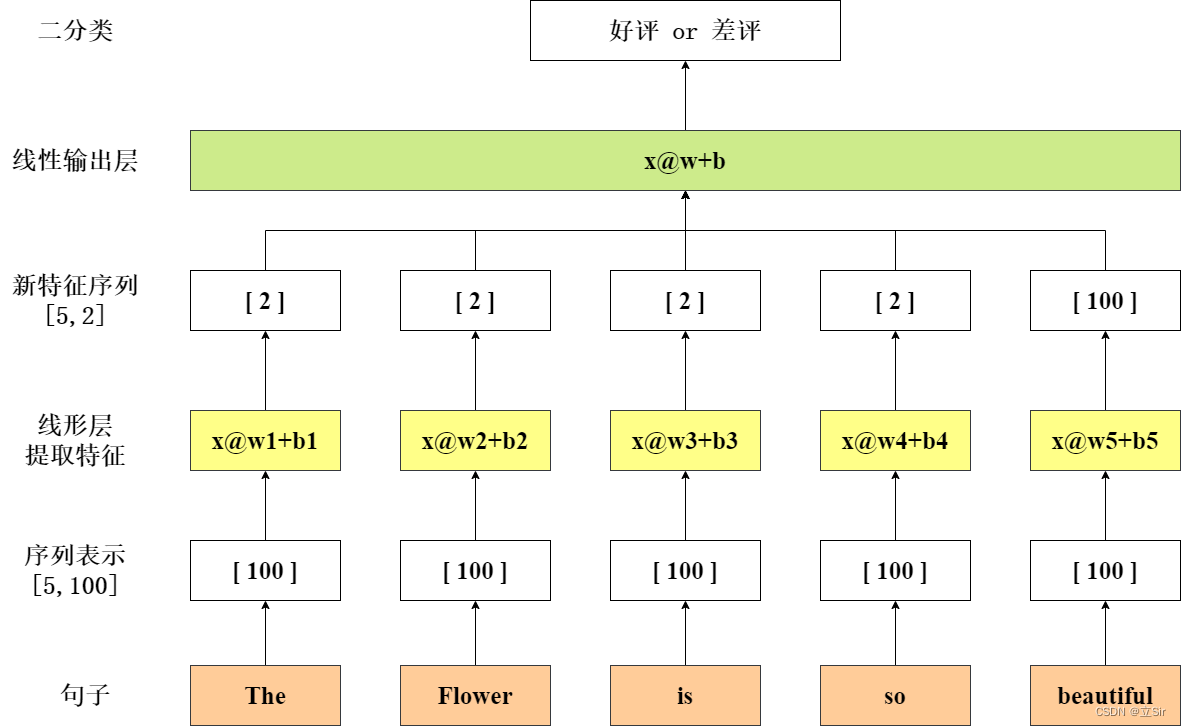现在有一个句子 The flower is so beautiful 作为输入，通过 wordembedding 每个单词用一个长度为 100 的向量来表示，然后将每个单词输入至线性层提取特征，每个单词的输出结果是一个长度为 2 的向量，最后将所有单词聚合起来，经过一个线性层输出得到分类结果。

传统的序列处理方法存在许多缺陷：

（1）计算量庞大。现实生活中的单词量巨大，对每个单词生成一个线性层 x@w+b 提取特征，然后再对线性层输出结果做聚合，模型非常复杂，参数量极其庞大。

（2）没有考虑上下文语境。传统方法只是针对一句话中的每个单词做单独的分析，没有联系前后单词之间的信息。如：i do not think the flower is beautiful 句子中，不能看到 beautiful 就说这句话一定是好评，要联系到上文的 not 再做分析。

# 2. RNN 原理解析

针对传统序列任务模型存在的问题，RNN做出了改进：

（1）优化参数量。通过权值共享，把每个单词的 w1、w2、w3... 用一个张量 W 来表示，一个RNN层就处理一整个句子。

（2）联系上下文语境。使用一个时序单元处理上下文信息，当前时刻的输入一定要考虑到上一时刻的输出。

下面仍以语言的情感分析任务为例，向大家介绍RNN的基本原理。

RNN单元的计算公式为：

其中，​ 代表当前时刻的输入特征​ 代表上一时刻的输出，也是上一时刻聚合后的语境信息；

接下来把公式展开：

其中，​ 代表对当前时刻输入的特征提取，​ 代表对之前语境信息的特征提取，然后对计算结果使用 tanh 激活函数，得到本时刻更新后的语境信息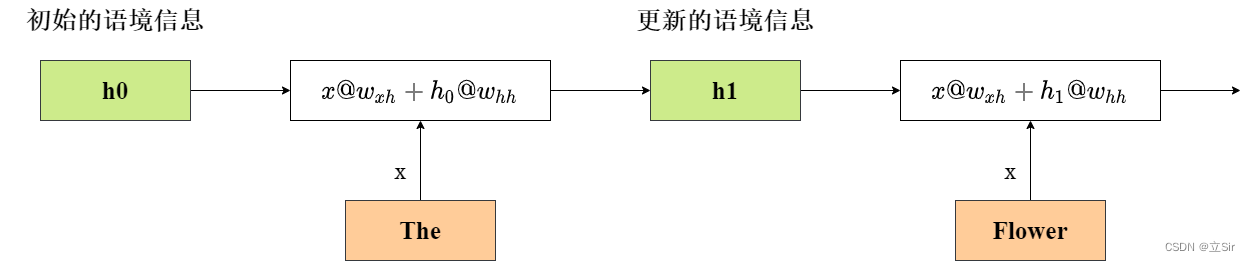# 3. RNN 的梯度推导

下面以时间序列预测任务为例，向大家介绍一下 RNN 的梯度更新方式，如下图。

取 RNN 层的最后一个语境信息 ht 作为预测结果输出。predict 代表前向传播得出的预测值target 代表真实值损失函数为预测值和真实值的均方误差MSE

前向传播：

线性变换：

损失函数：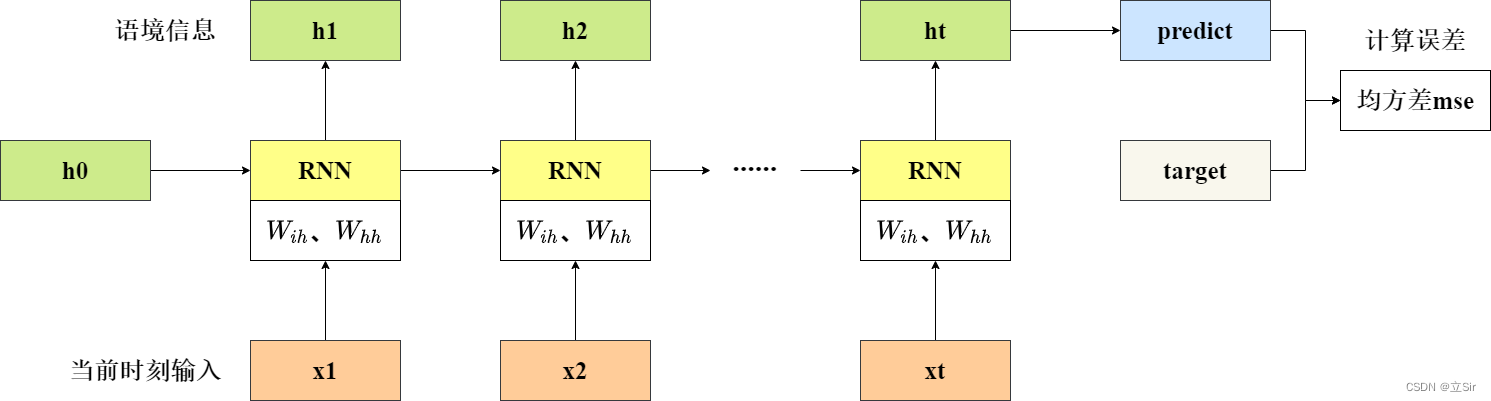通过损失函数值更新每个时刻的语境的梯度信息

反向传播公式：

分别对每个分式计算偏微分：

其中：

# 4. 模型结构

下面向大家介绍一下 RNN 层的结构，各个输入和输出张量的 shape

首先，网络输入的 shape 为 [seq_len, batch, feature_len]。其中 seq_len 代表特征的个数batch 代表有多少个句子feature_len 代表每个特征的向量表示hidden_len 代表 RNN 单元的隐含层神经元个数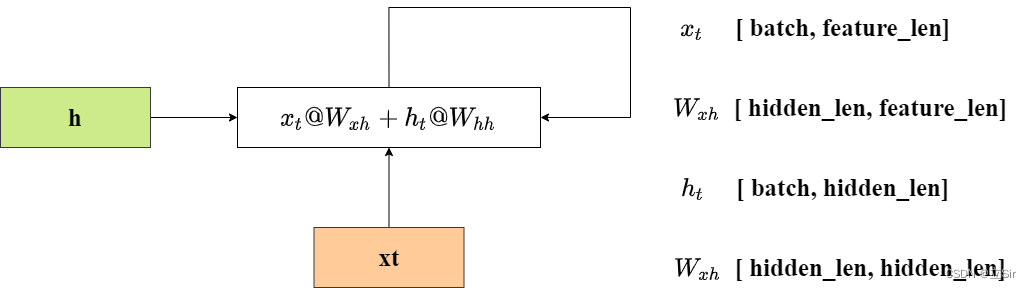以 batch=3，seq_len=10，feature_len=100，hidden_len=20 为例，向大家介绍网络的输入和输出的特征的 shape 变化

RNN 层的公式：

shape 变化为：

带入具体数值：

下面在Pytorch中展示单个RNN层的参数的shape

import torch
from torch import nn

# 100代表feature_len每个单词的向量表示的长度
# 20代表hidden_len经过RNN层之后每个单词的向量表示长度变成20
rnn = nn.RNN(100, 20)

# 查看RNN单元的参数
print(rnn._parameters.keys())

# 查看每个参数的shape
print('W_xh:', rnn.weight_ih_l0.shape,
'bias_xh:', rnn.bias_ih_l0.shape,
'W_hh:', rnn.weight_hh_l0.shape,
'bias_hh:', rnn.bias_hh_l0.shape)

'''
输出结果：

odict_keys(['weight_ih_l0', 'weight_hh_l0', 'bias_ih_l0', 'bias_hh_l0'])

W_xh: torch.Size([20, 100])
bias_xh: torch.Size()
W_hh: torch.Size([20, 20])
bias_hh: torch.Size()
'''

# 5. Pytorch 代码实现

## 5.1 单层 RNN 实现

首先需要实例化一个RNN层

input_size：用多少长的向量来表示一个单词。

hidden_size：经过RNN层特征提取后 ，每个单词用多少长的向量表示。

num_layers：共有多少层RNN。

rnn = nn.RNN(input_size, hidden_size, num_layers)

前向传播函数

x：当前时刻的输入特征，shape = [seq_len, batch, feature_len]

h0：上一时刻的语境信息，shape = [num_layers, batch, hidden_size]

out：最后一个时刻的输出结果，shape = [seq_len, batch, hidden_len]

h：所有时刻的语境状态，shape = [num_layers, batch, hidden_size]

out, h = rnn(x, h0)

以 batch=3，seq_len=10，feature_len=100，hidden_len=20 为例，单个RNN层的代码如下：

import torch
from torch import nn

# input_size：代表每个单词的向量表示的长度
# hidden_size：代表特征提取后，每个单词的向量表示长度
# num_layers：代表RNN的层数
rnn = nn.RNN(input_size=100, hidden_size=20, num_layers=1)  # 实例化单层的RNN层

# 构造输入层shape=[seq_len, batch, feature_len]
x = torch.randn(10, 3, 100)

# 构造上一时刻的语境shape=[num_layers, batch, hidden_size]
h0 = torch.randn(1, 3, 20)

# 前向传播的返回值如下
# out：代表每个时刻的h的输出结果shape=[seq_len, batch, hidden_len]
# h：代表最后一个时刻的输出结果shape=[num_layers, batch, hidden_size]
out, h = rnn(x, h0)

print('out:', out.shape, 'h:', h.shape)

'''
输出结果
out: torch.Size([10, 3, 20])
h: torch.Size([1, 3, 20])
'''

## 5.2 多层 RNN 实现

参数和上面相同，这里要注意的就是在前向传播的输出结果中，h 代表在最后一个时刻上看之前的所有语境信息，而 out 代表每个RNN层的输出结果

4层的RNN代码如下：

import torch
from torch import nn

# input_size：代表每个单词的向量表示的长度
# hidden_size：代表特征提取后，每个单词的向量表示长度
# num_layers：代表RNN的层数
rnn = nn.RNN(input_size=100, hidden_size=20, num_layers=4)  # 实例化4层的RNN层

# 构造输入层shape=[seq_len, batch, feature_len]
x = torch.randn(10, 3, 100)

# 构造初始时刻的语境shape=[num_layers, batch, hidden_size]
h0 = torch.randn(4, 3, 20)

# out：代表每个时刻的h的输出结果shape=[seq_len, batch, hidden_len]
# h：代表最后一个时刻的输出结果shape=[num_layers, batch, hidden_size]
out, h = rnn(x, h0)

print('out:', out.shape, 'h:', h.shape)
'''
out: torch.Size([10, 3, 20])
h: torch.Size([4, 3, 20])
'''

## 5.3 单层 RNNCell 实现

nn.RNN将所有句子全部都输入至RNN层中，而 nn.RNNCell 需要手动输入每个句子，并且当前时刻的输出状态不会自动进入到下一时刻。单个RNNCell结构如下。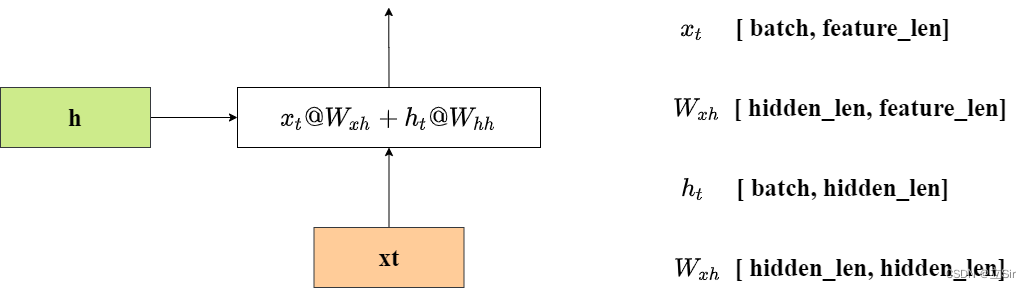实现过程如下：

import torch
from torch import nn

# input_size：代表每个单词的向量表示的长度
# hidden_size：代表特征提取后，每个单词的向量表示长度
rnncell = nn.RNNCell(input_size=100, hidden_size=20)  # 实例化单层的RNNcell层

# 构造输入层shape=[seq_len, batch, feature_len]
inputs = torch.randn(10, 3, 100)

# 构造初始时刻的语境shape=[batch, hidden_size]
h0 = torch.randn(3, 20)

# RNNCell的输入shape=[batch, feature_len]
for x in inputs:
# h0：代表当前时刻的语境信息shape=[batch, hidden_len]
h0 = rnncell(x, h0)

print('h0:', h0.shape)
'''
h0: torch.Size([3, 20])
'''

## 5.4 多层的 RNNCell 实现

以两层的 RNNCell 实现为例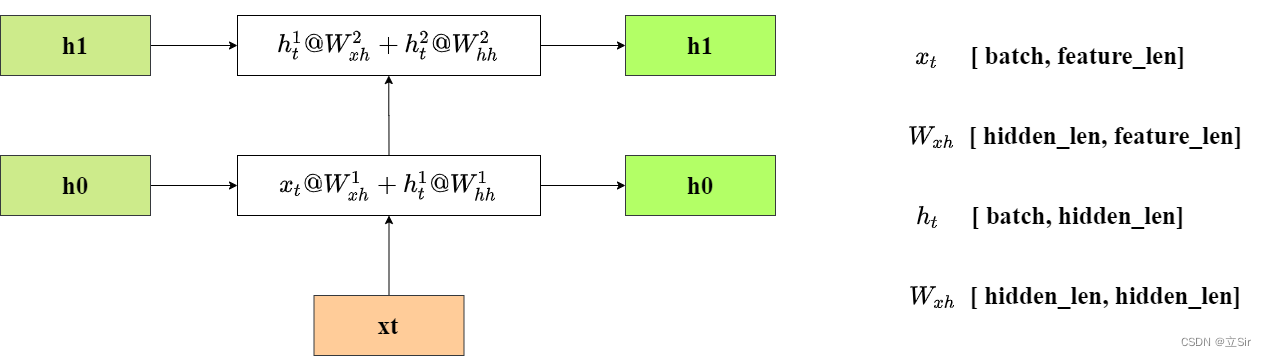第一个 RNNCell 层将每个单词的向量表示长度从 100 变成 20，第二个 RNNCell 层将每个单词的向量表示长度从 20 变成 15。

第一个 RNNCell 的输入当前时刻的单词和上一时刻的语境状态h0第二个 RNNCell 的输入第一个 RNNCell 的输出和上一时刻的语境状态h1

代码实现如下：

import torch
from torch import nn

# input_size：代表每个单词的向量表示的长度
# hidden_size：代表特征提取后，每个单词的向量表示长度
rnncell1 = nn.RNNCell(input_size=100, hidden_size=20)  # 实例化单层的RNNcell层
rnncell2 = nn.RNNCell(input_size=20, hidden_size=15)

# 构造输入层shape=[seq_len, batch, feature_len]
inputs = torch.randn(10, 3, 100)

# 构造初始时刻的语境shape=[batch, hidden_size]
h0 = torch.randn(3, 20)
h1 = torch.randn(3, 15)

# RNNCell的输入shape=[batch, feature_len]
for x in inputs:
# h0：代表当前时刻的语境信息shape=[batch, hidden_len]
h0 = rnncell1(x, h0)
h1 = rnncell2(h0, h1)

print('h1:', h1.shape)
'''
h1: torch.Size([3, 15])
'''
展开全文深度学习 pytorch 自然语言处理
• 1. 循环神经网络 RNN 2. Q&A GPT3, BERT 都是基于Transformer模型的改进，目前也是最火的。 voice和image融合算法，用多模态模型。比如自动驾驶领域的运用。 参考 ......

# 1. 循环神经网络 RNN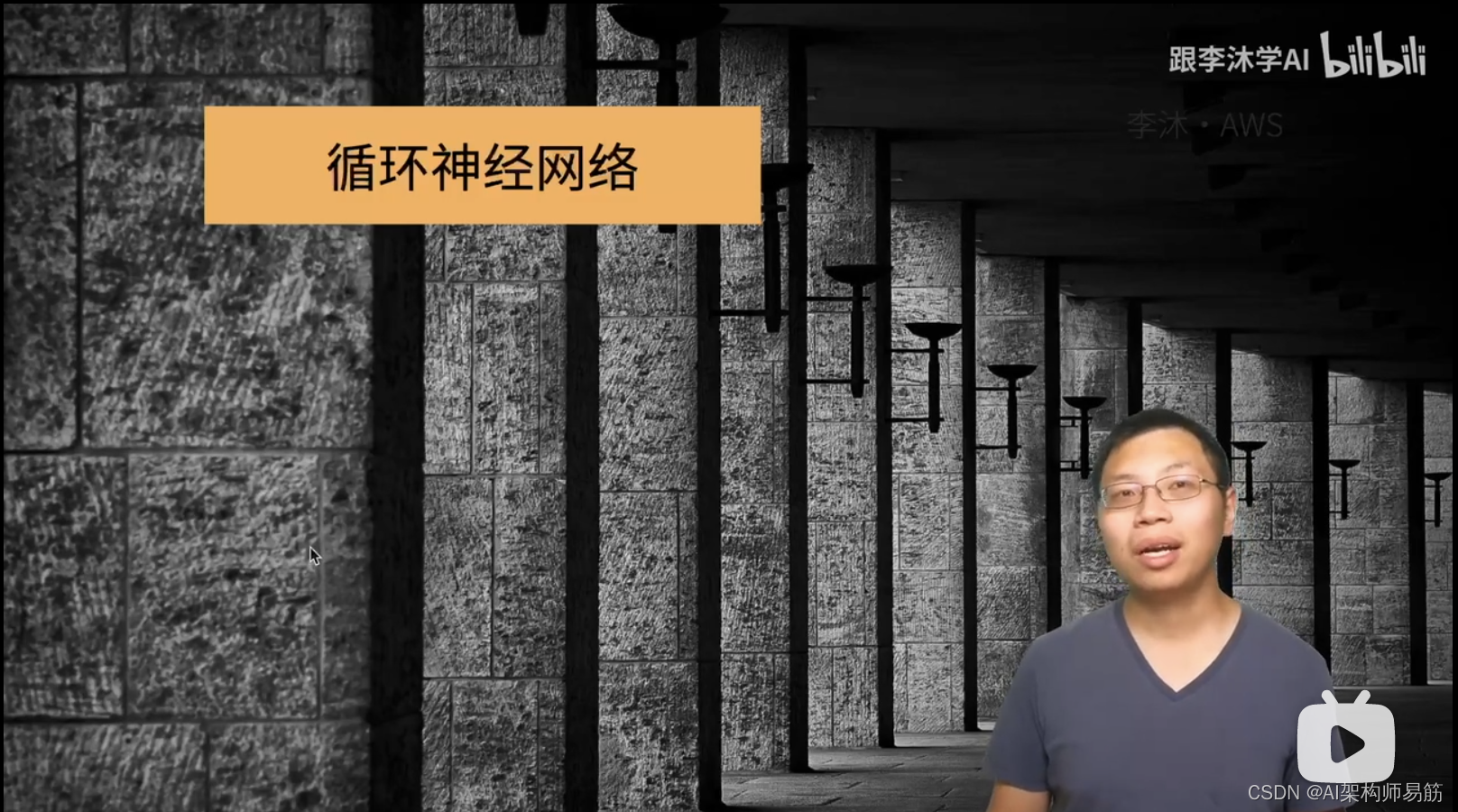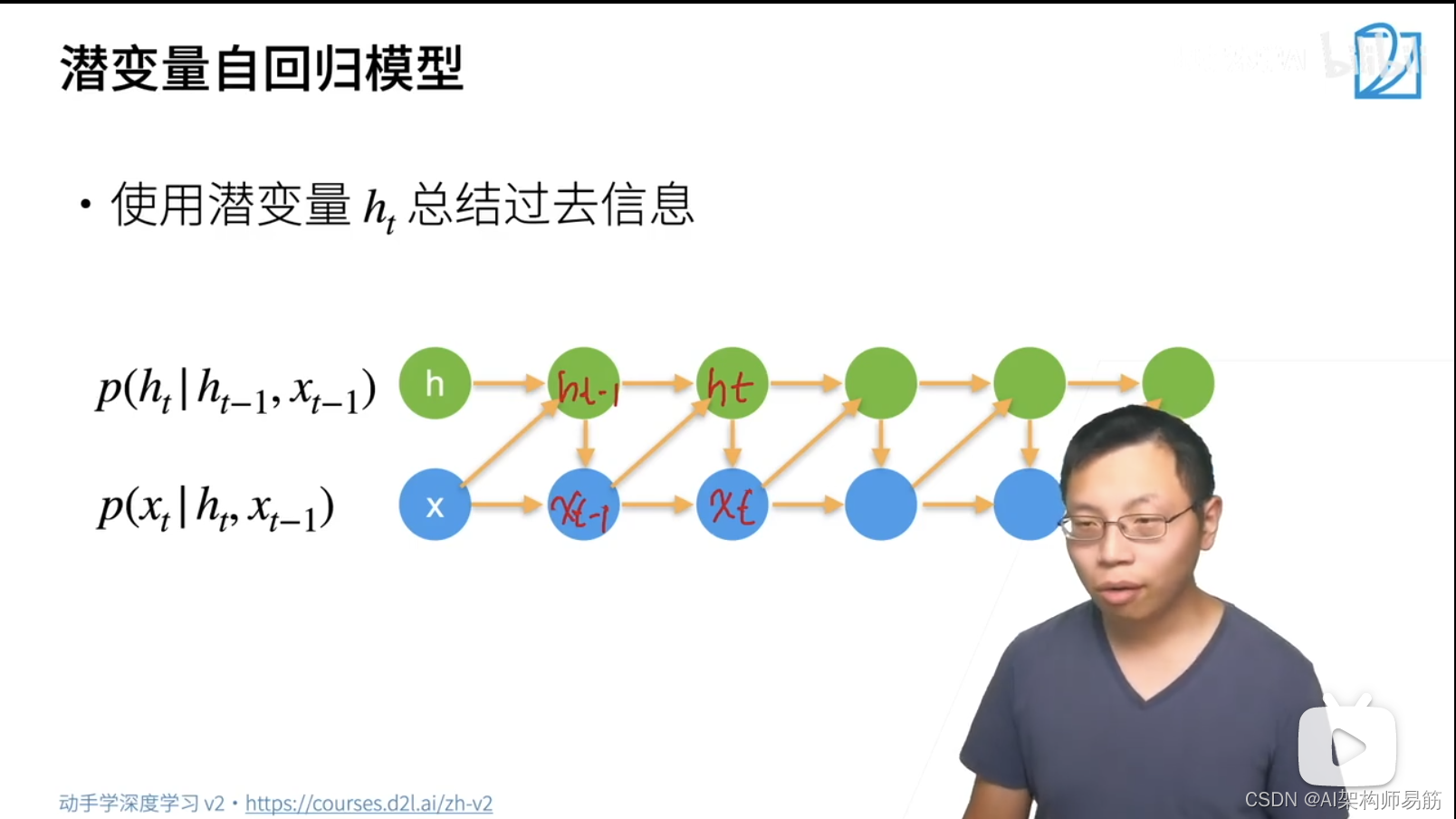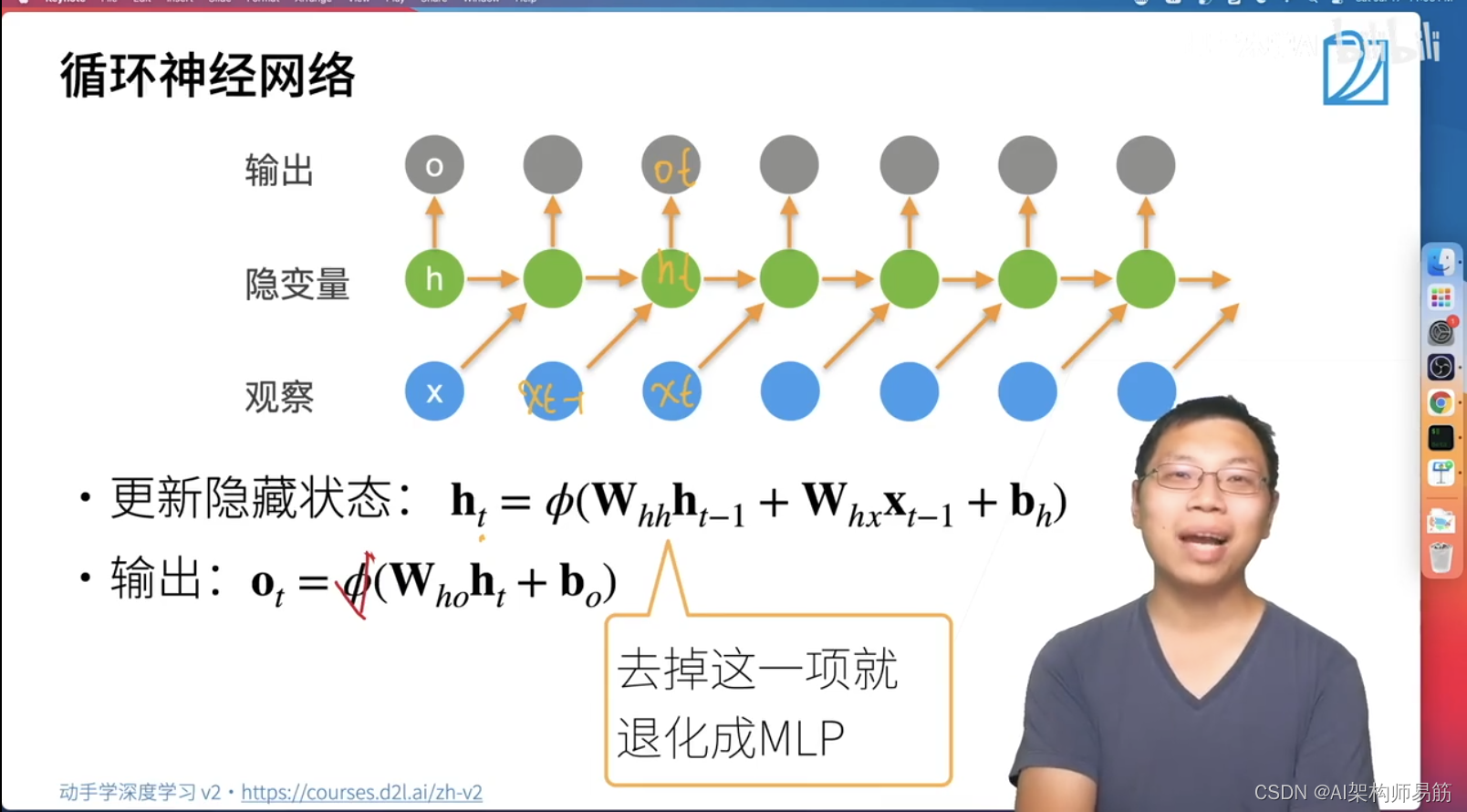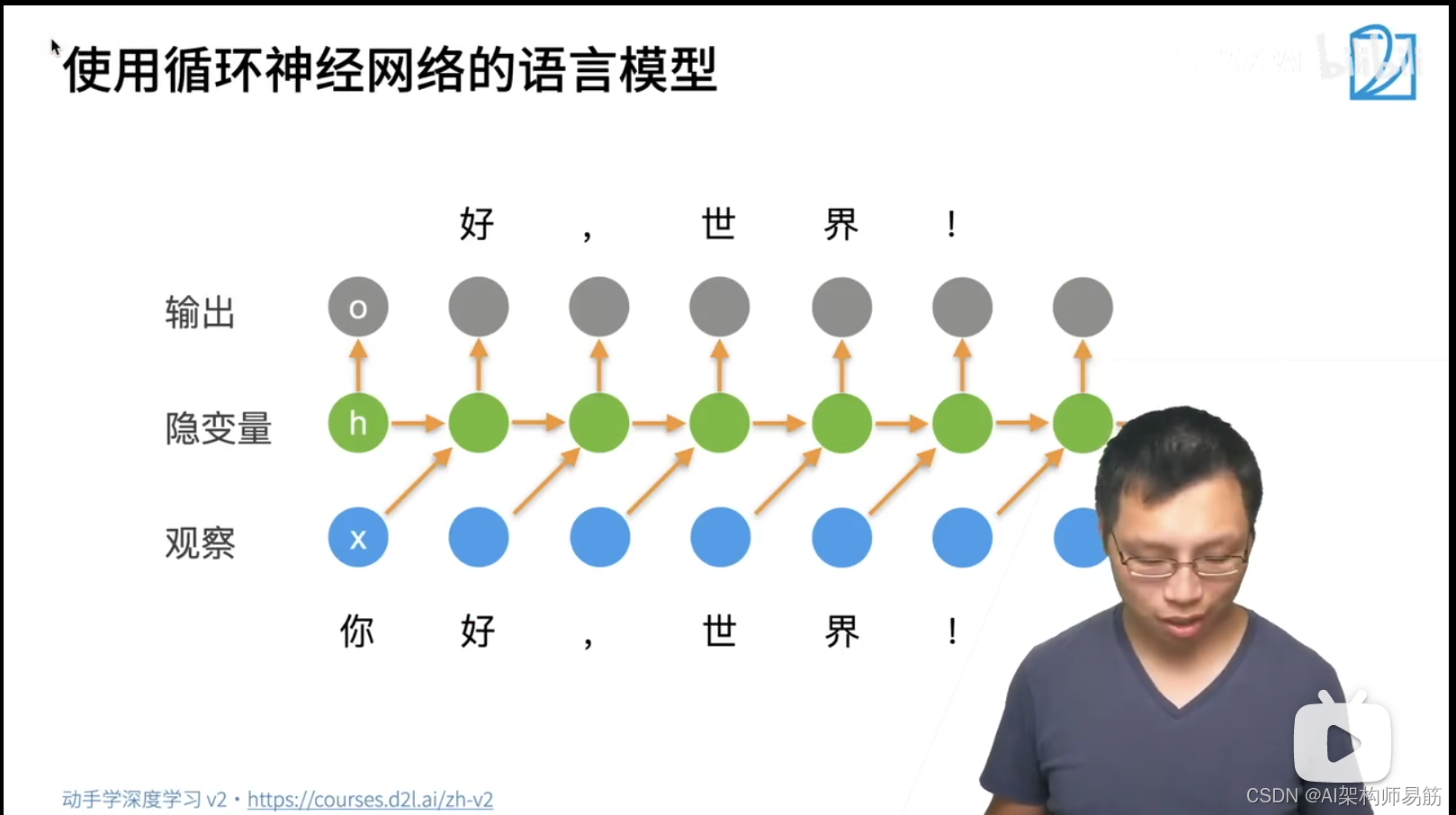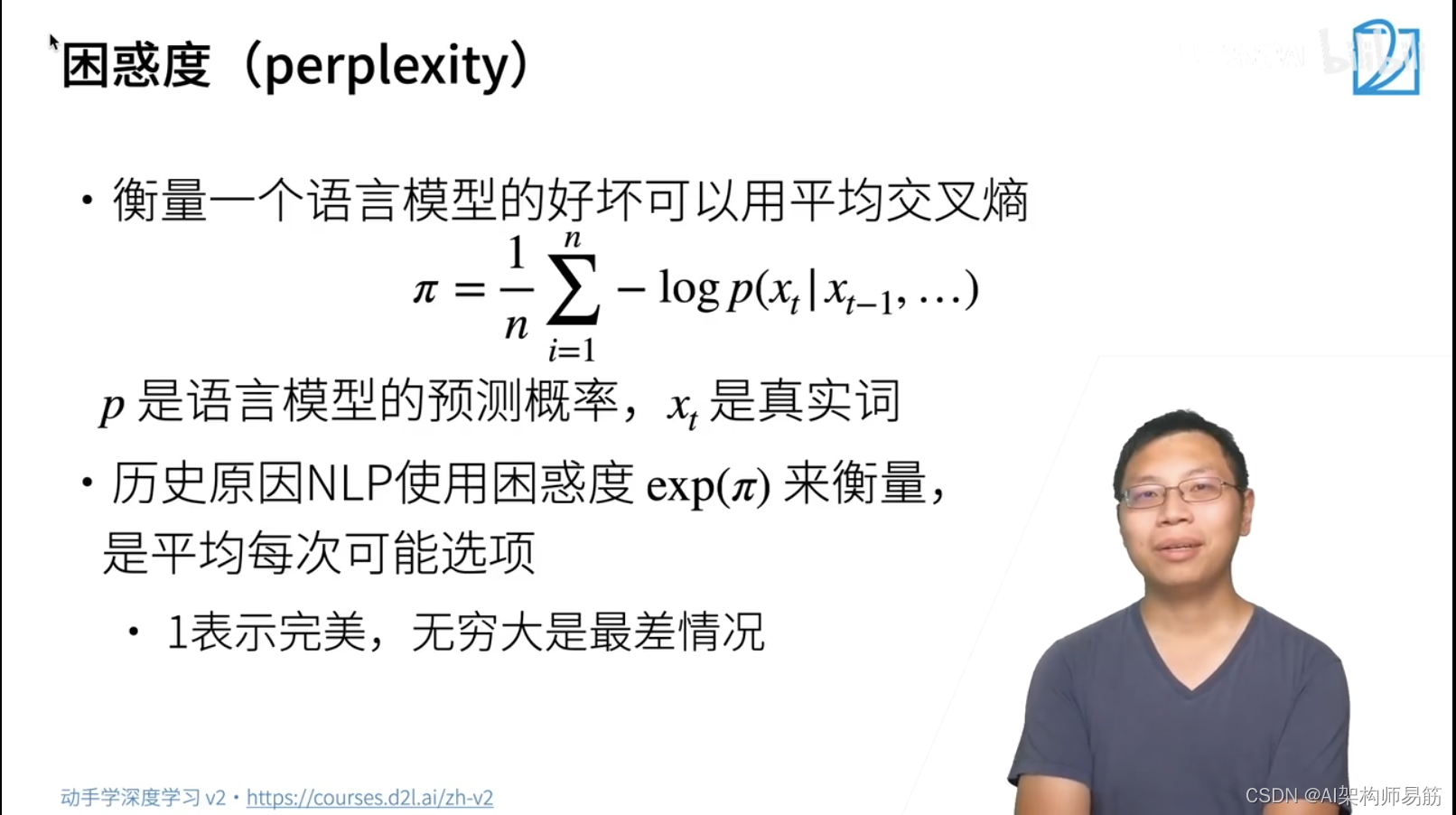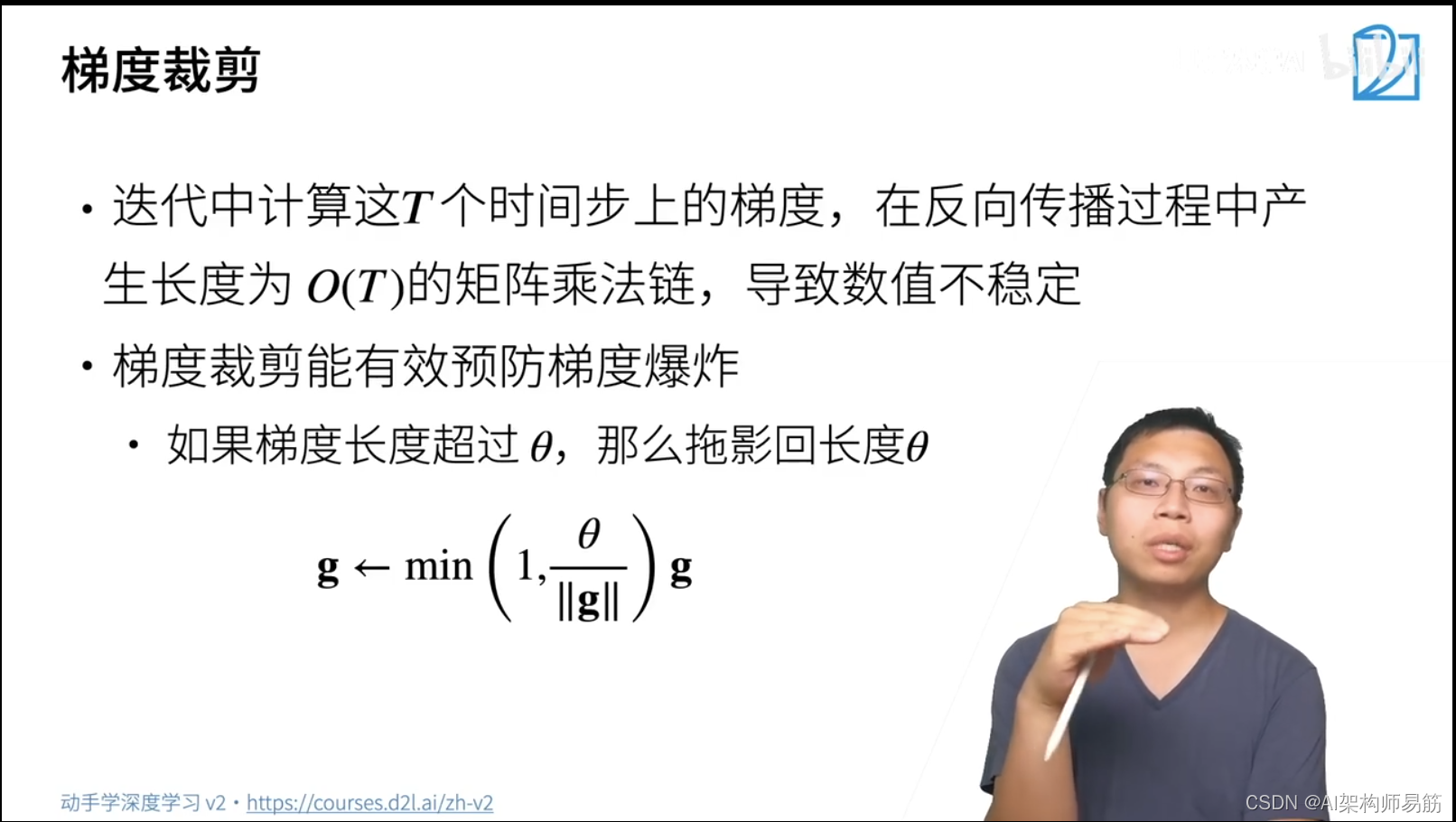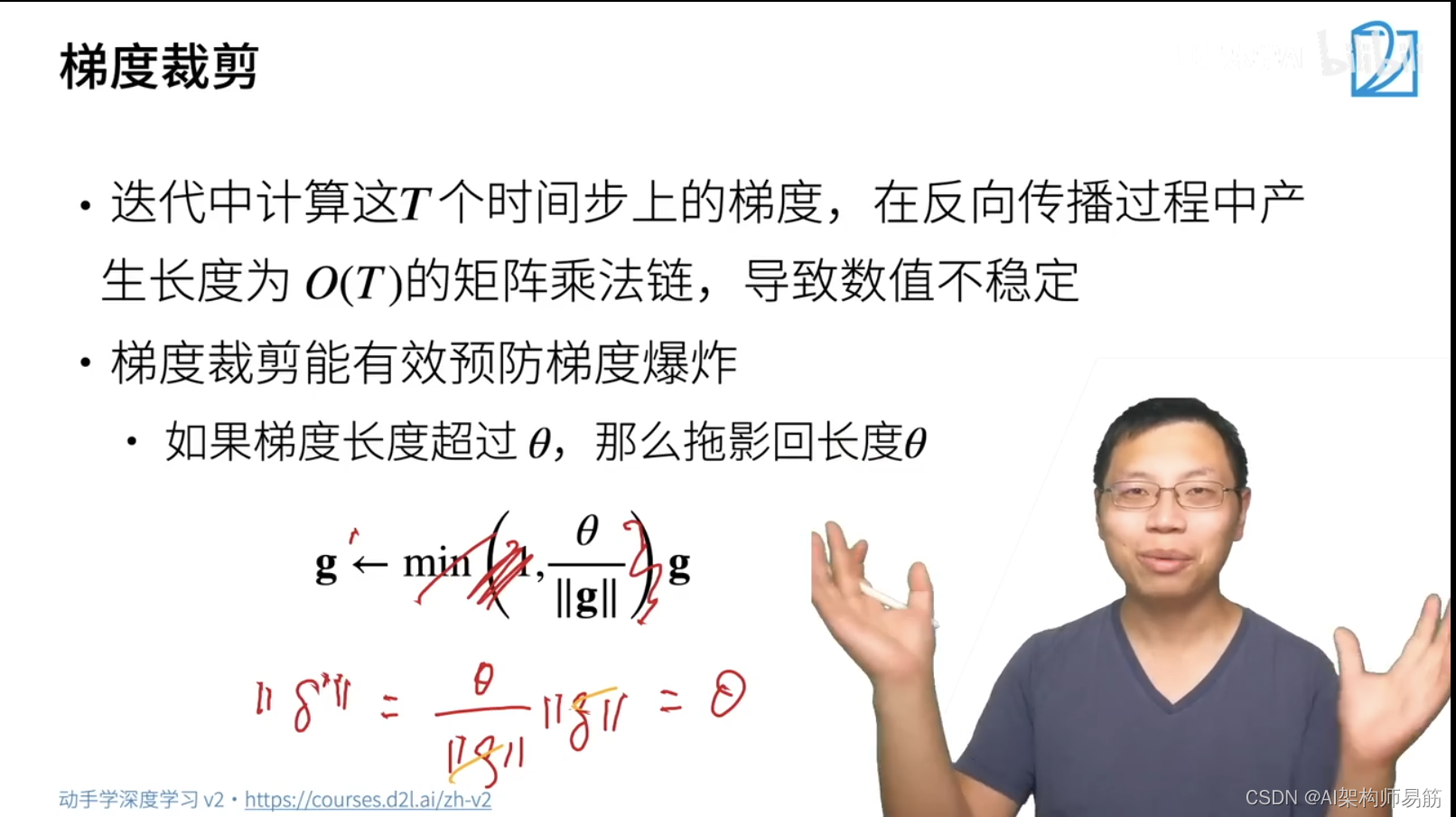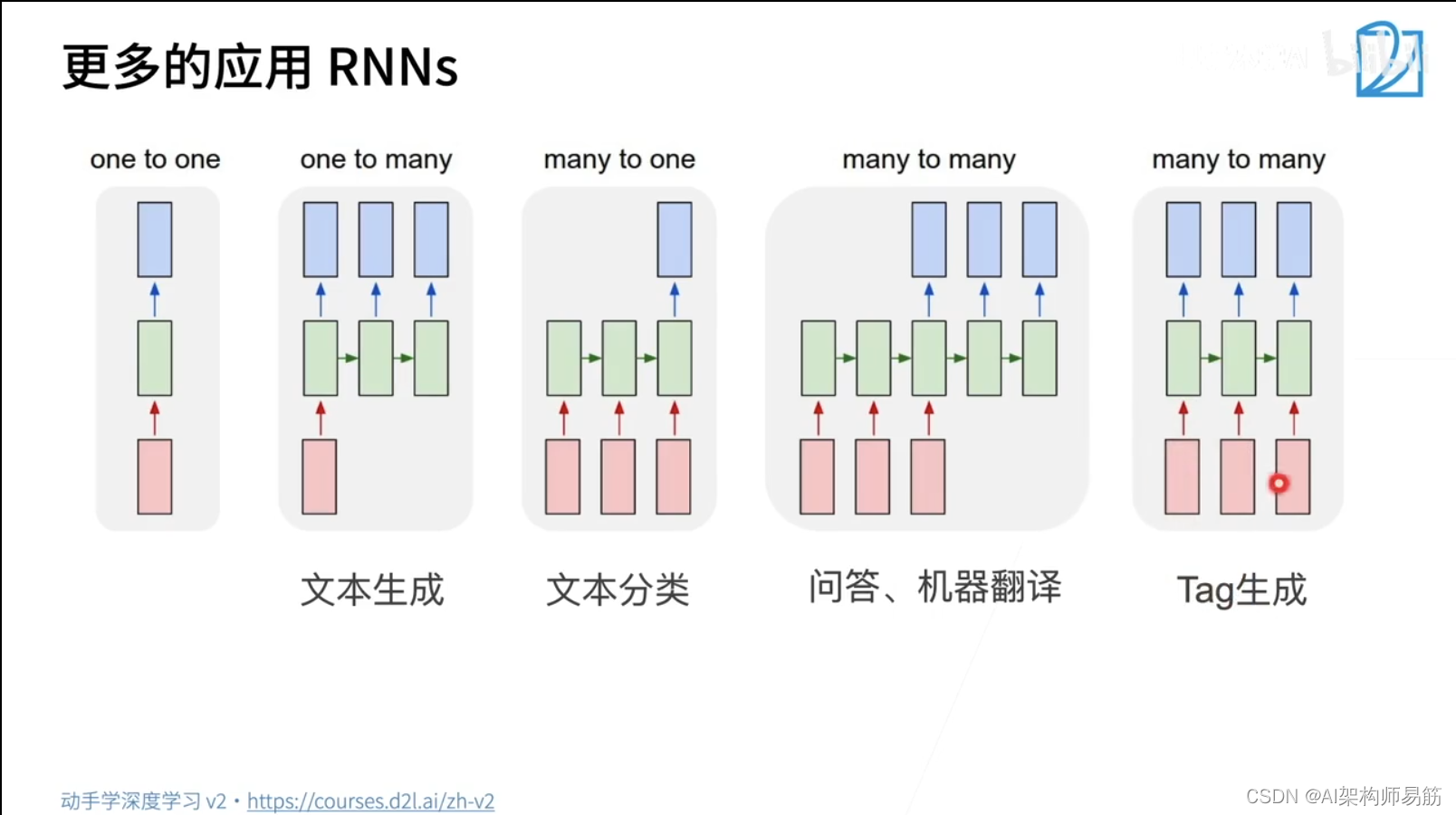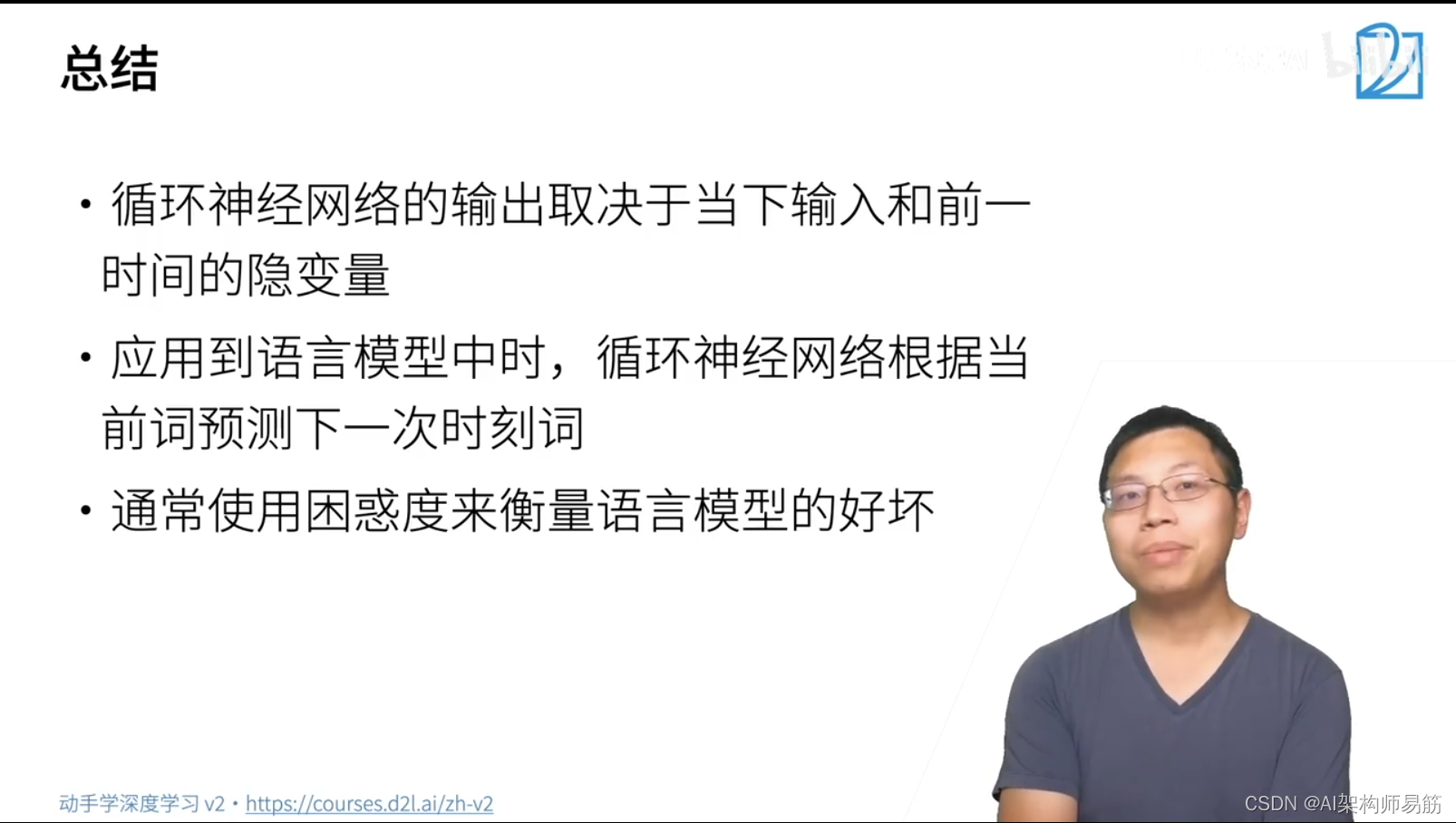# 2. 从零开始实现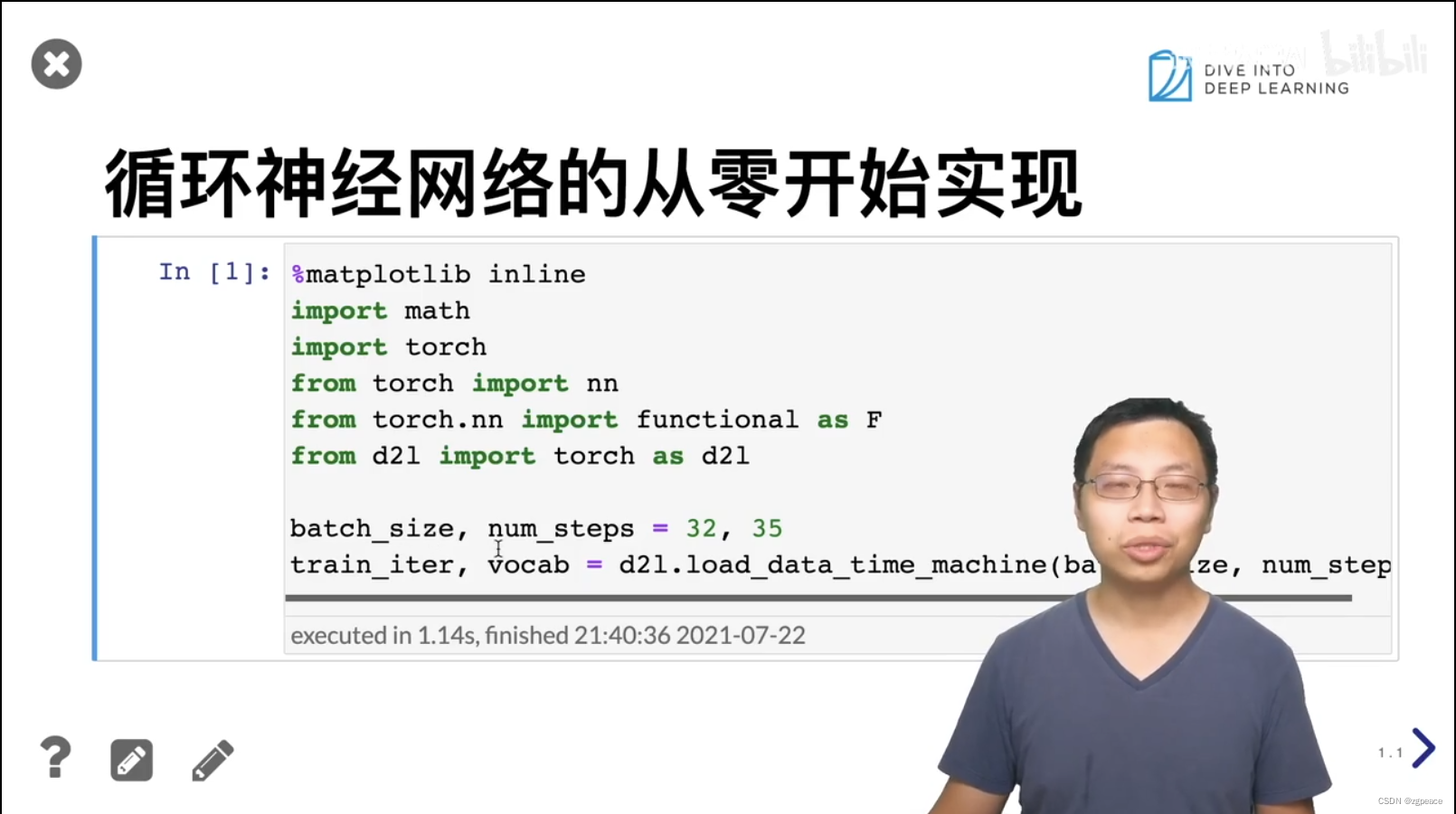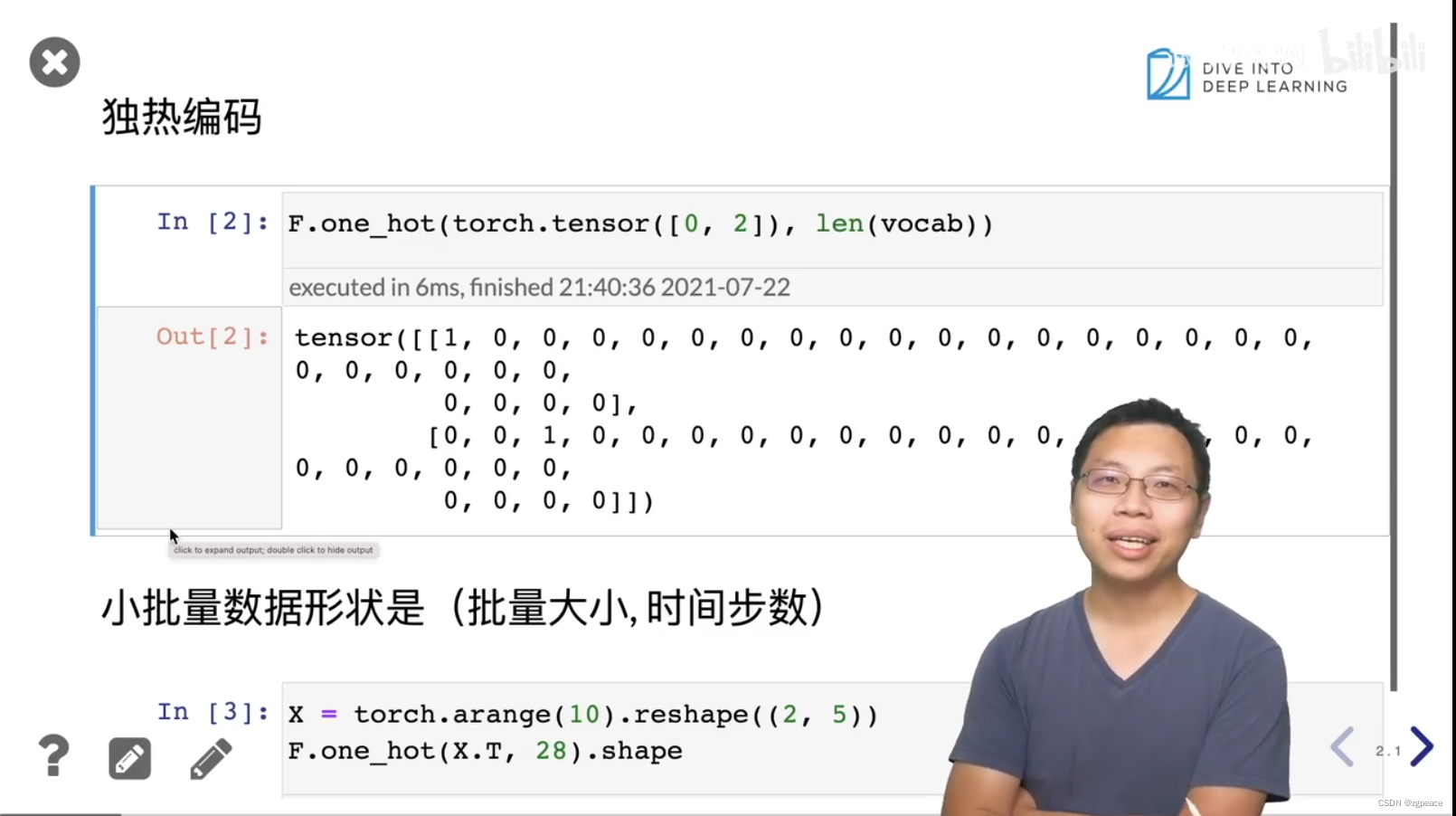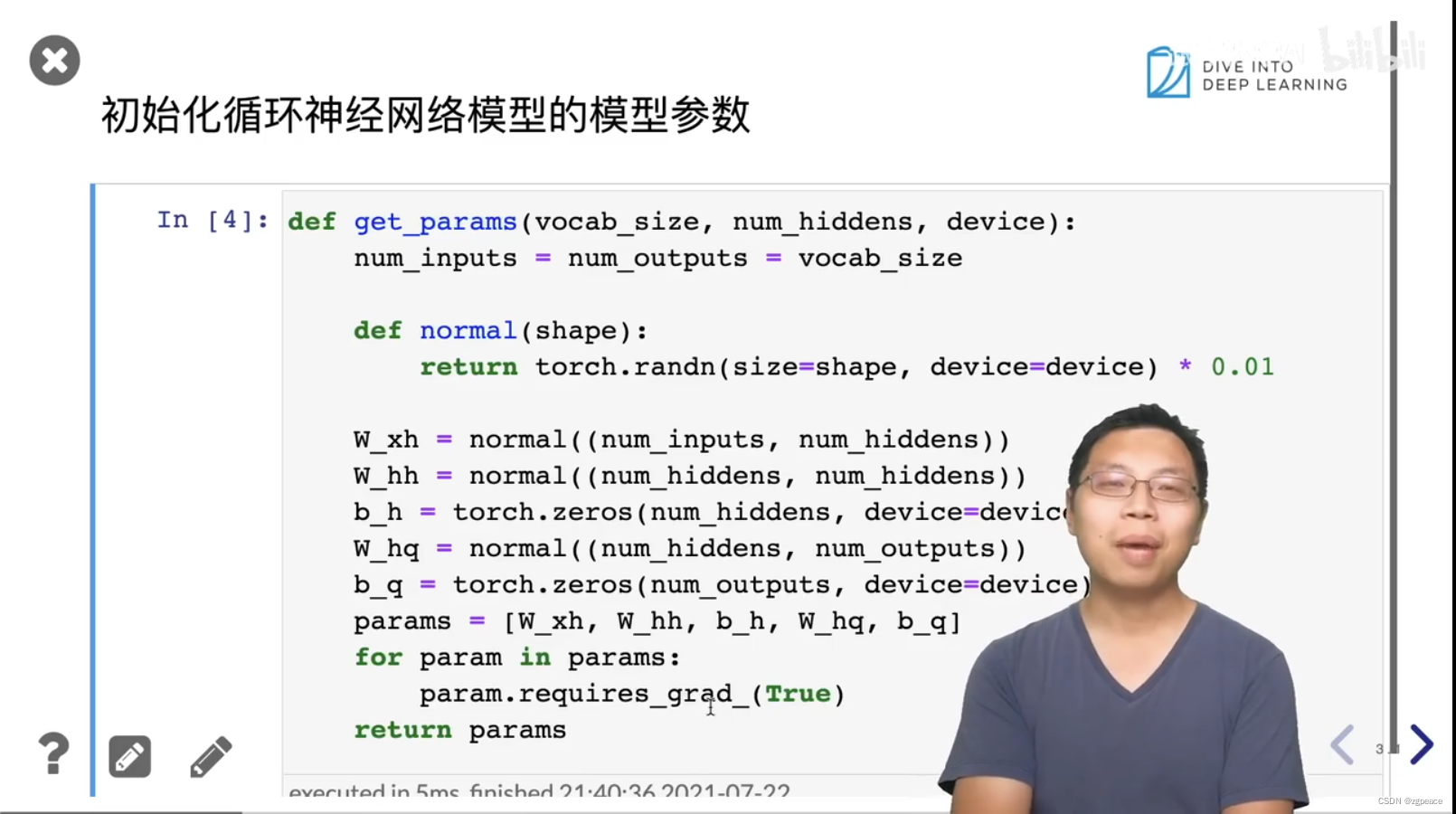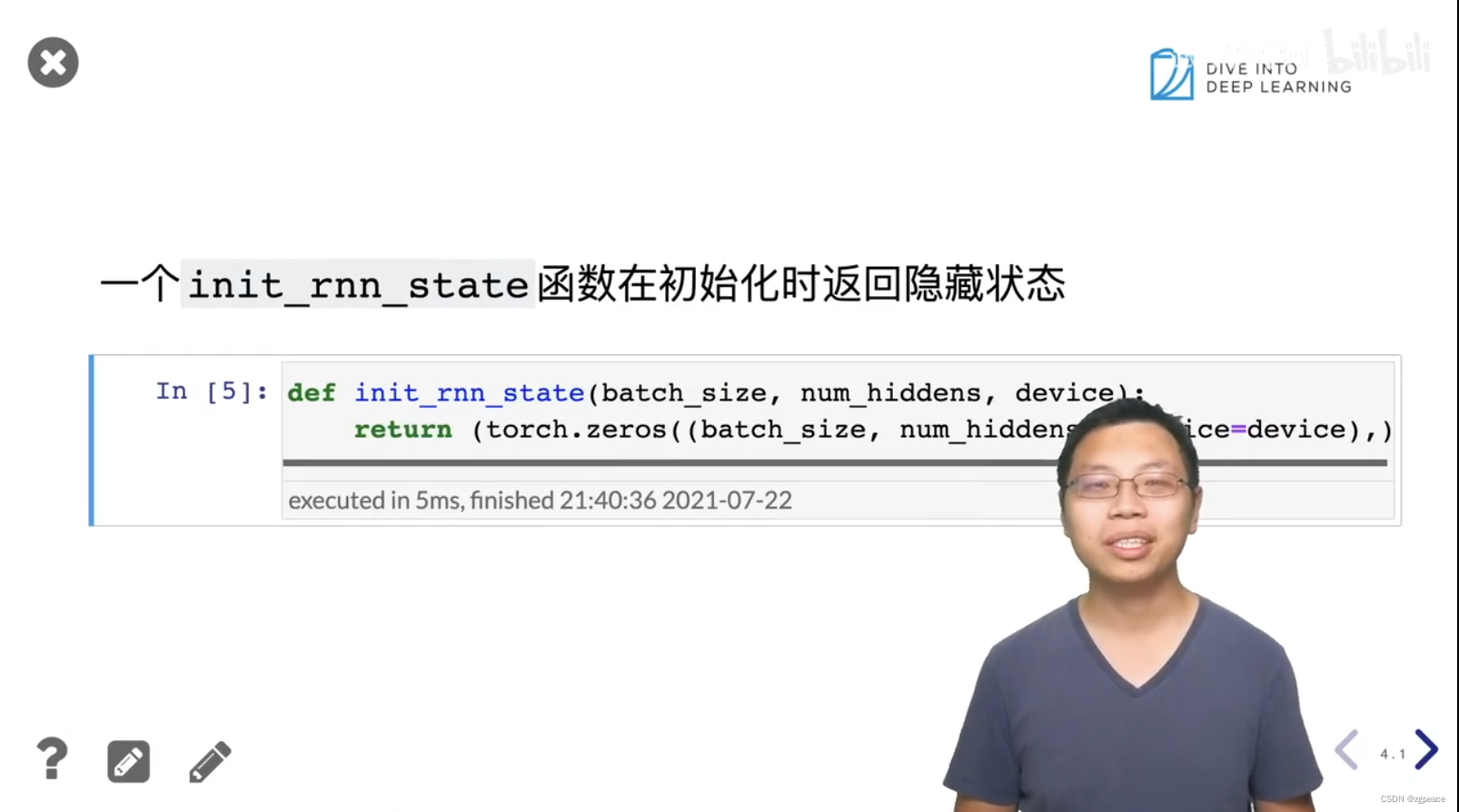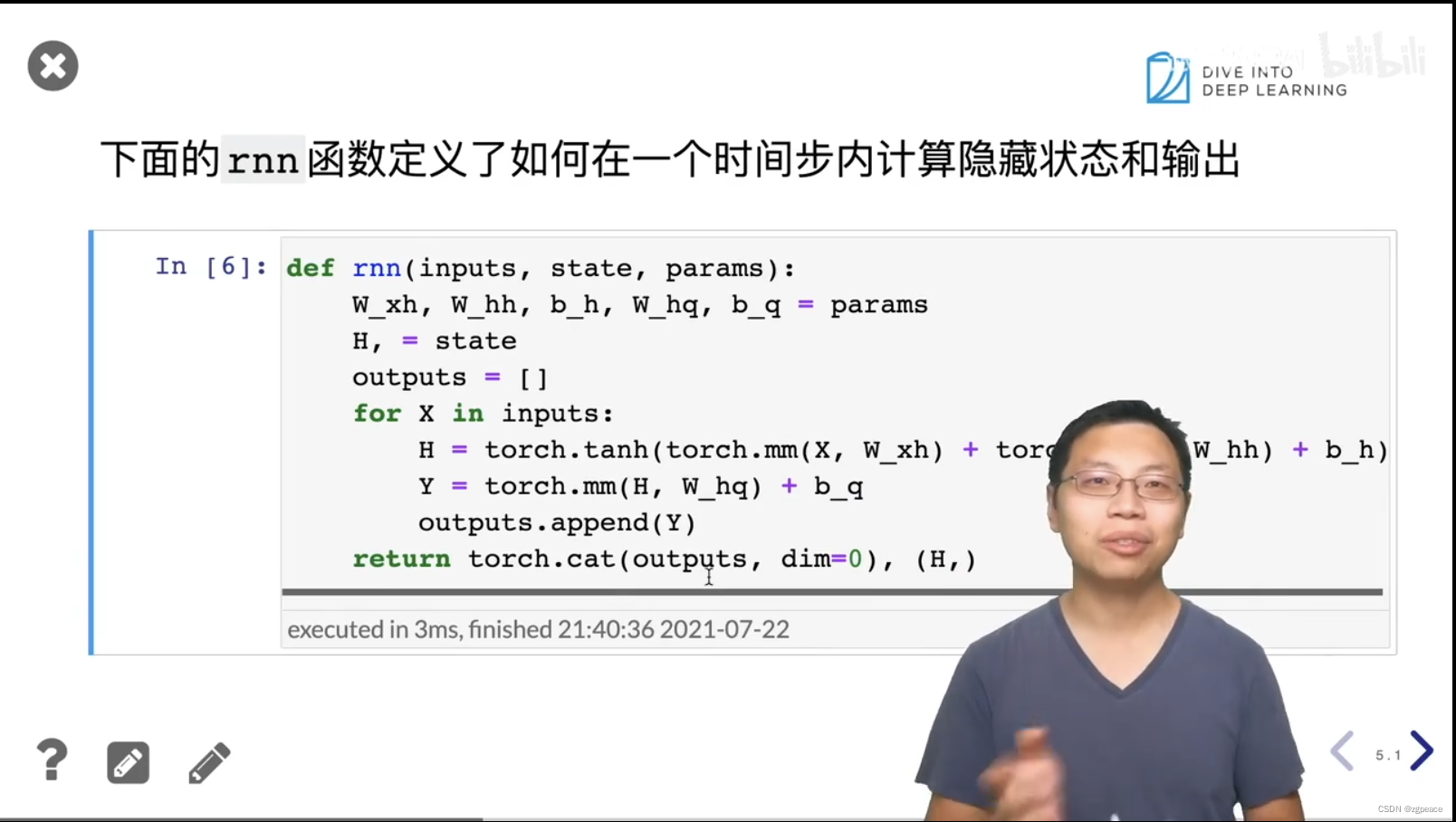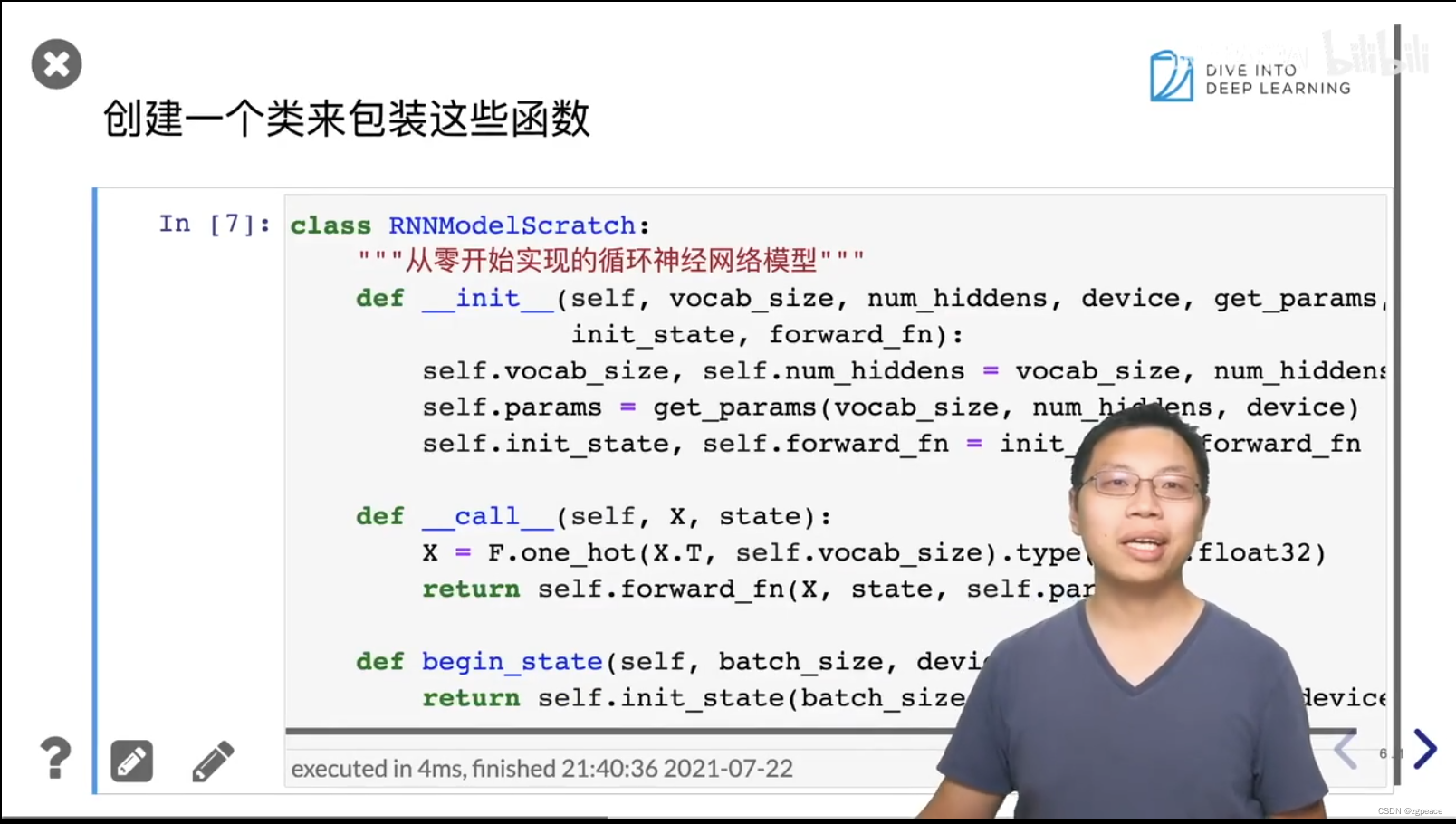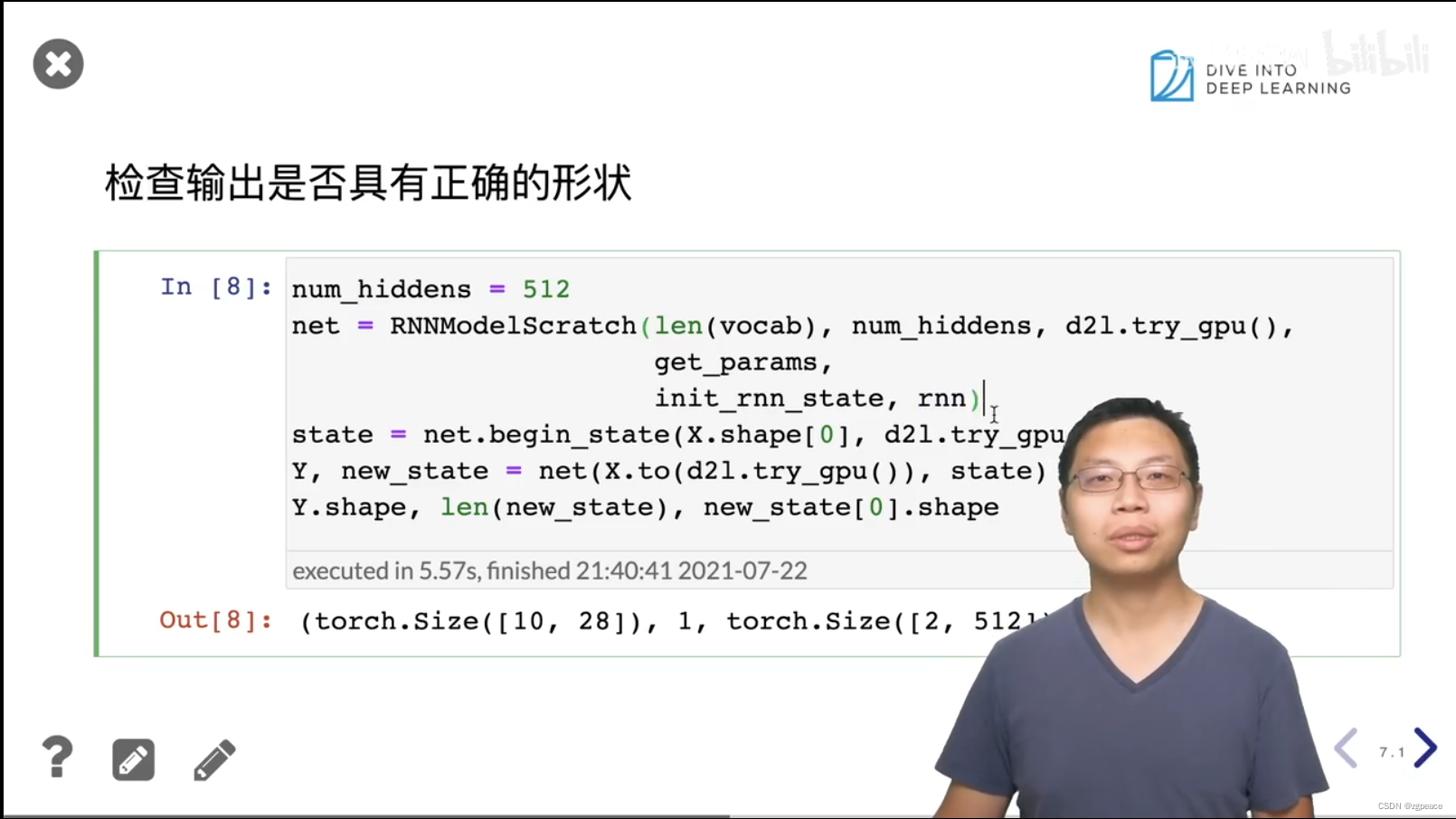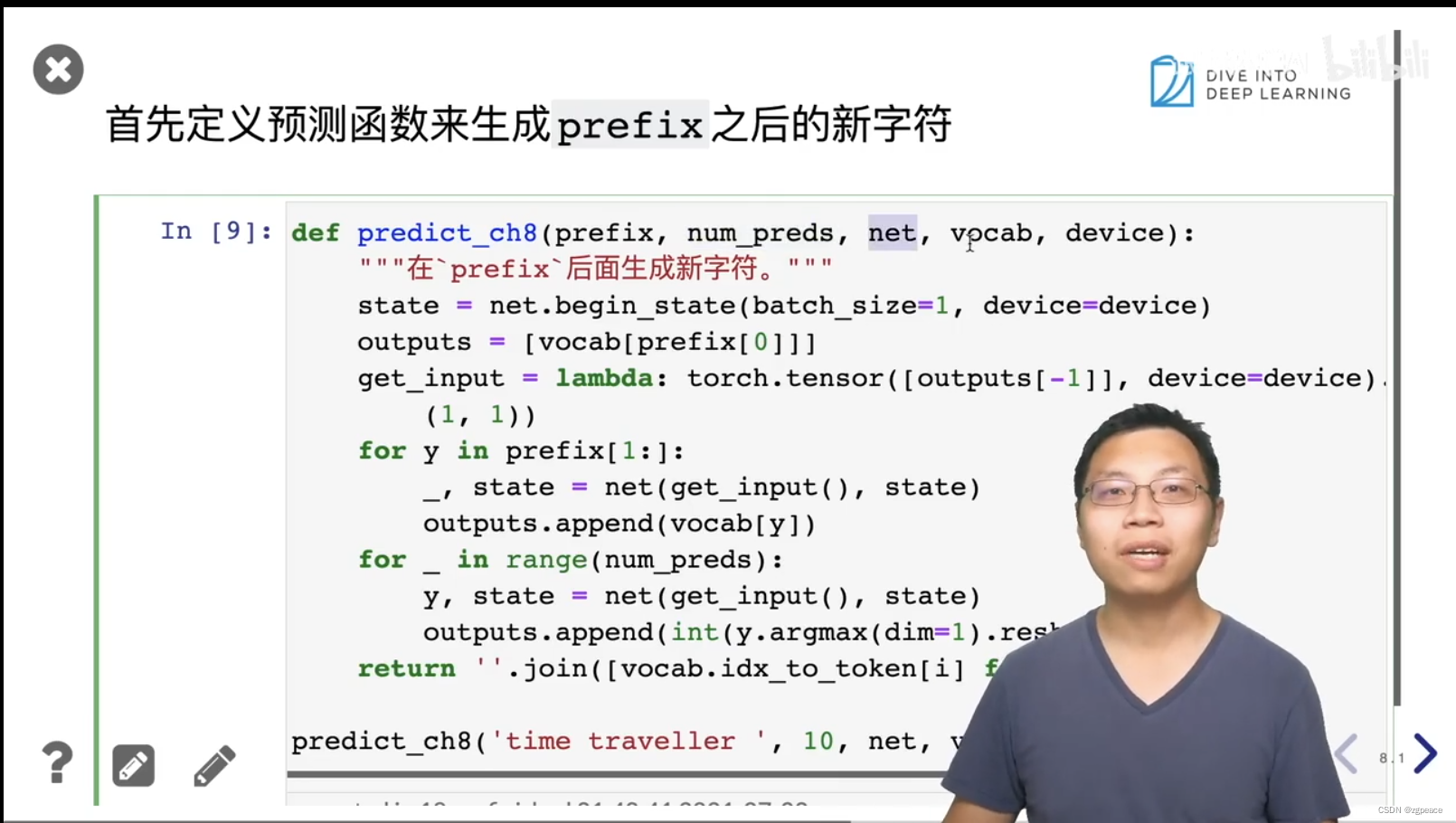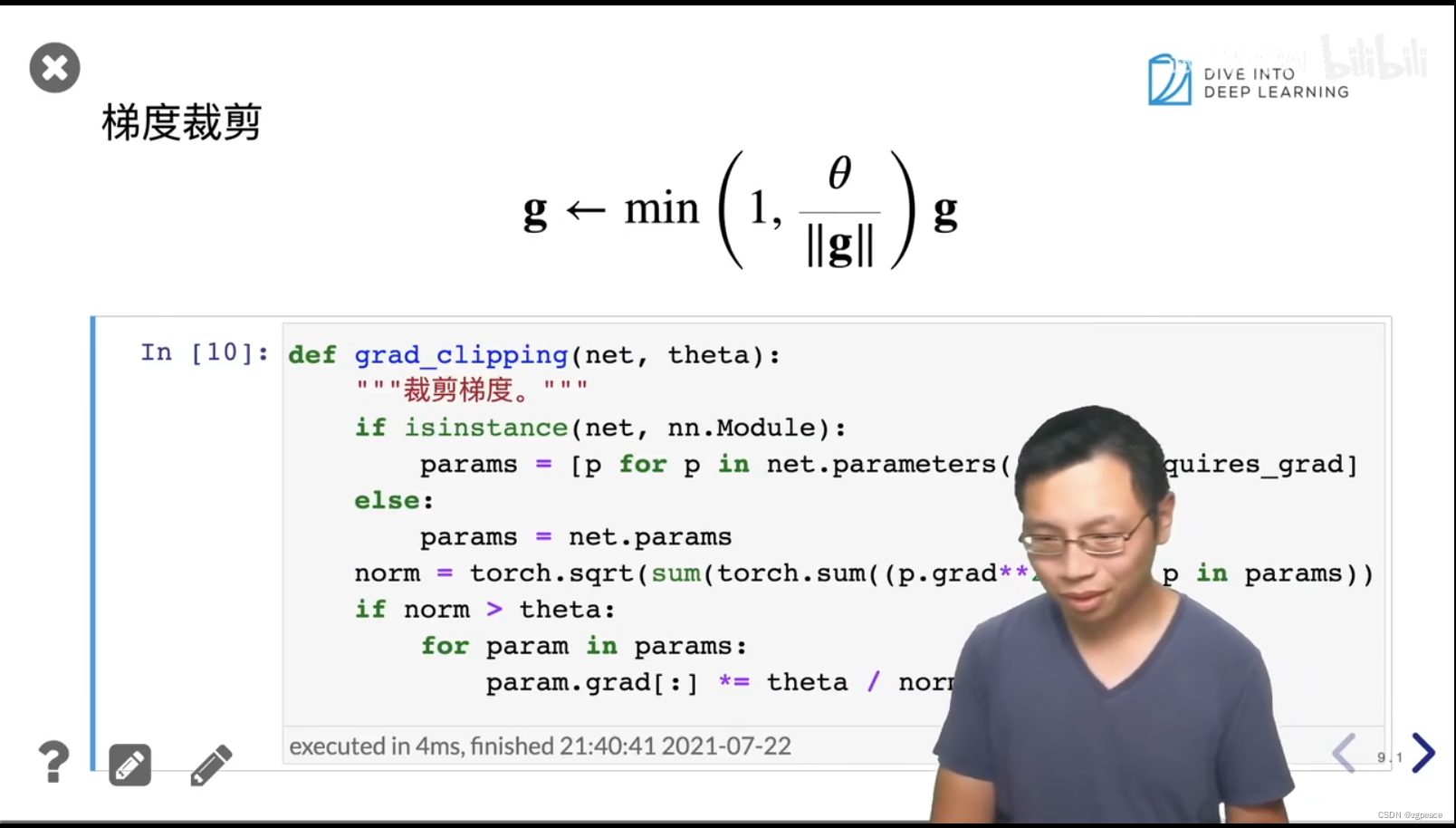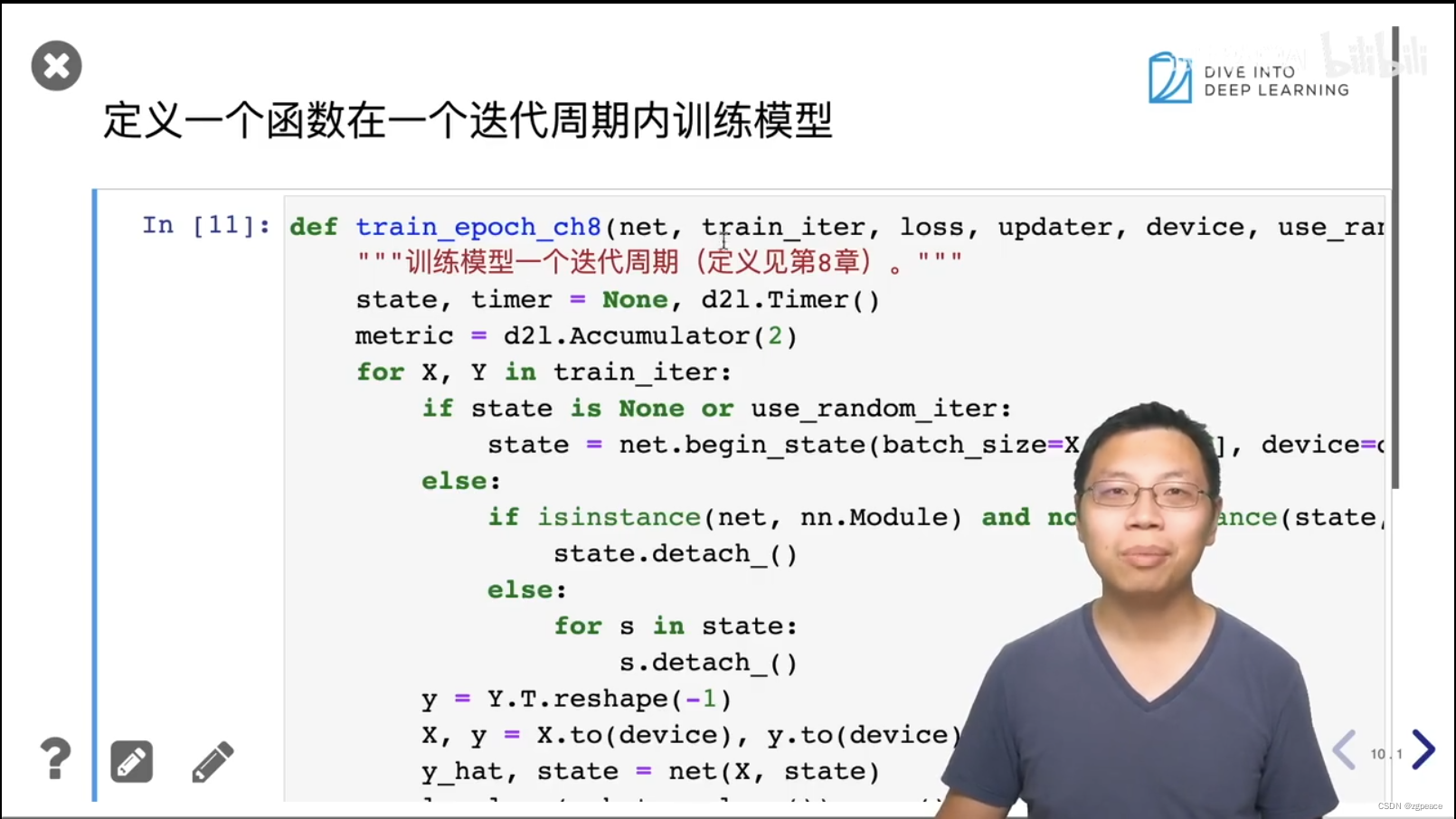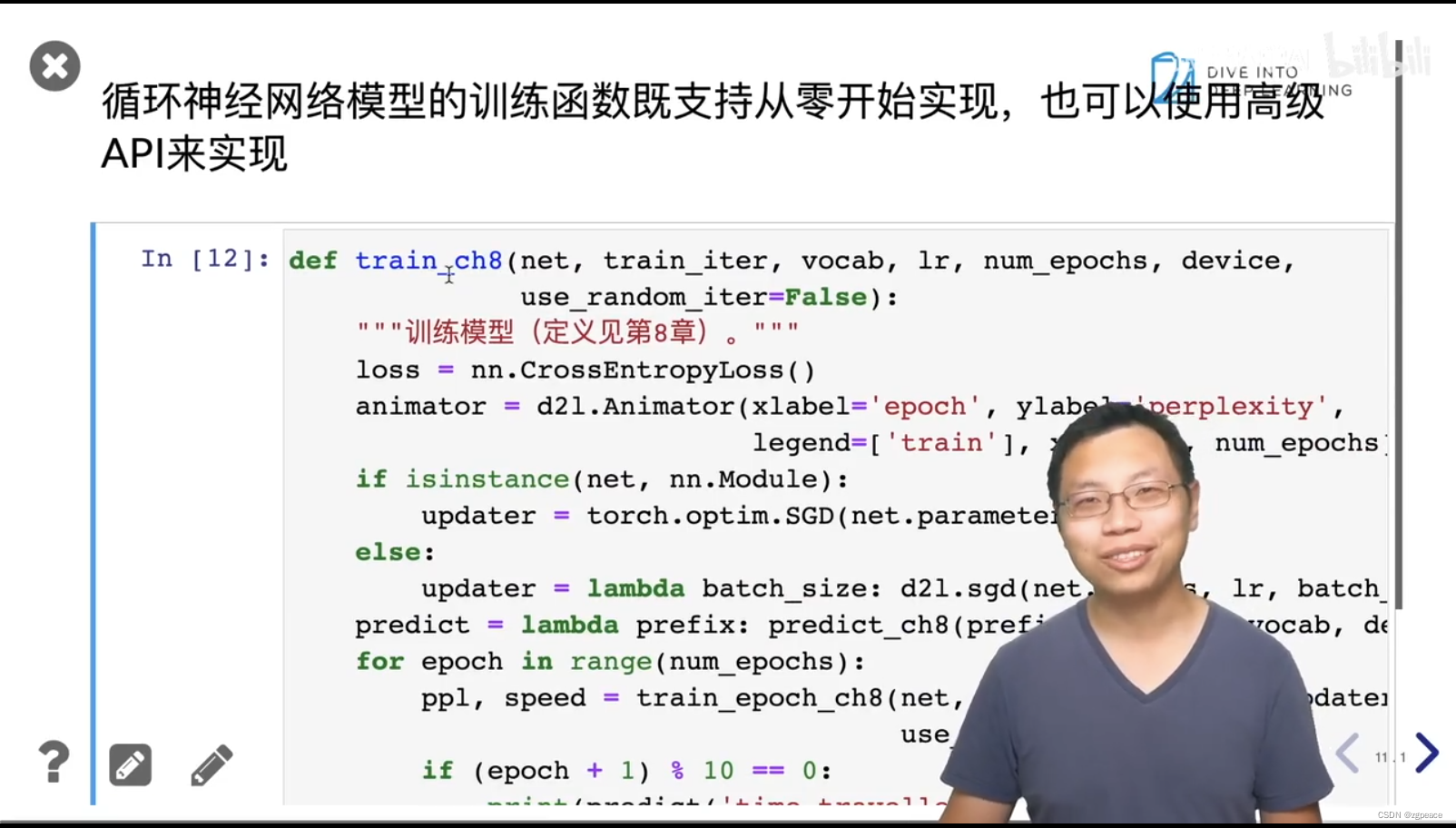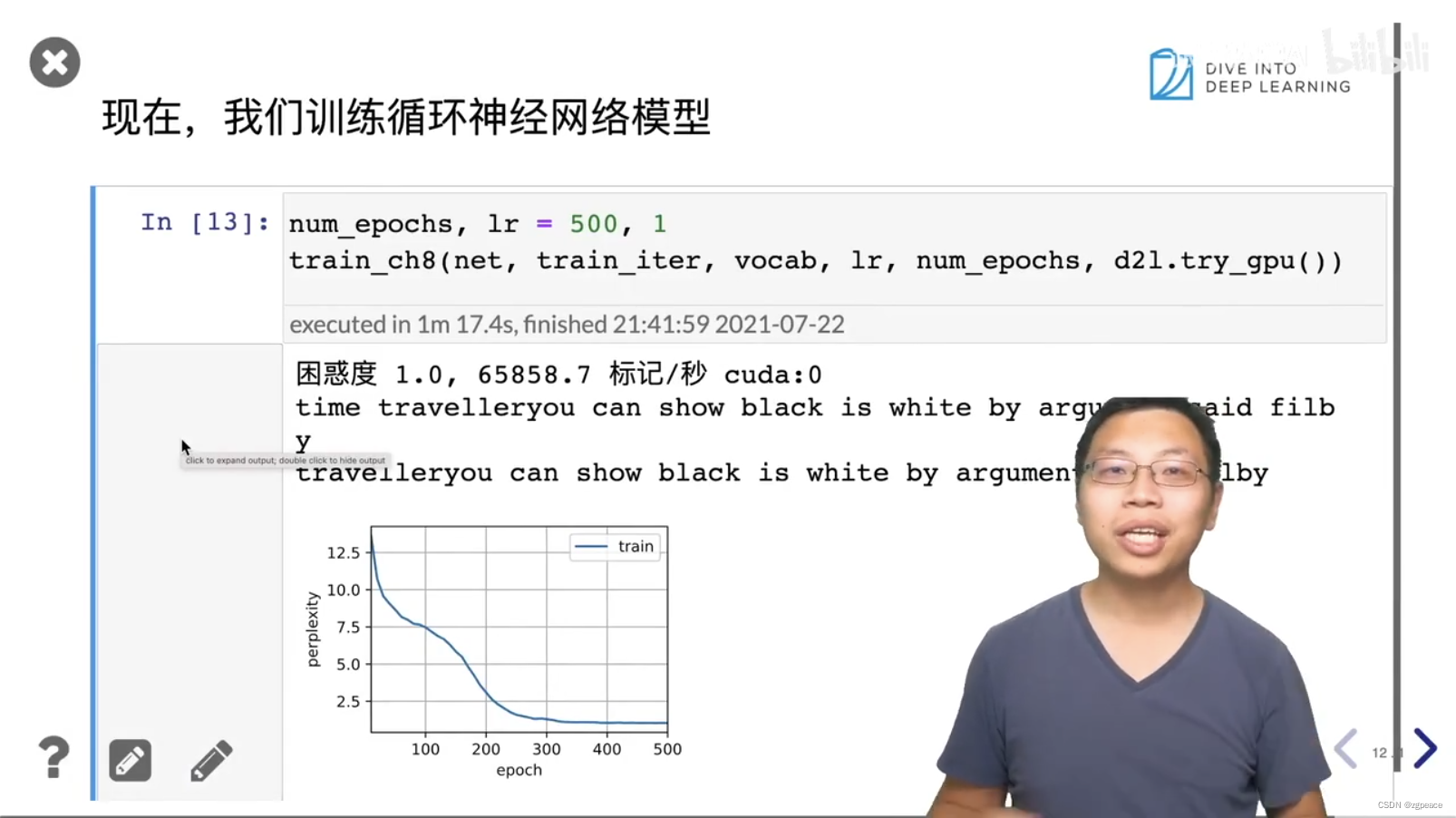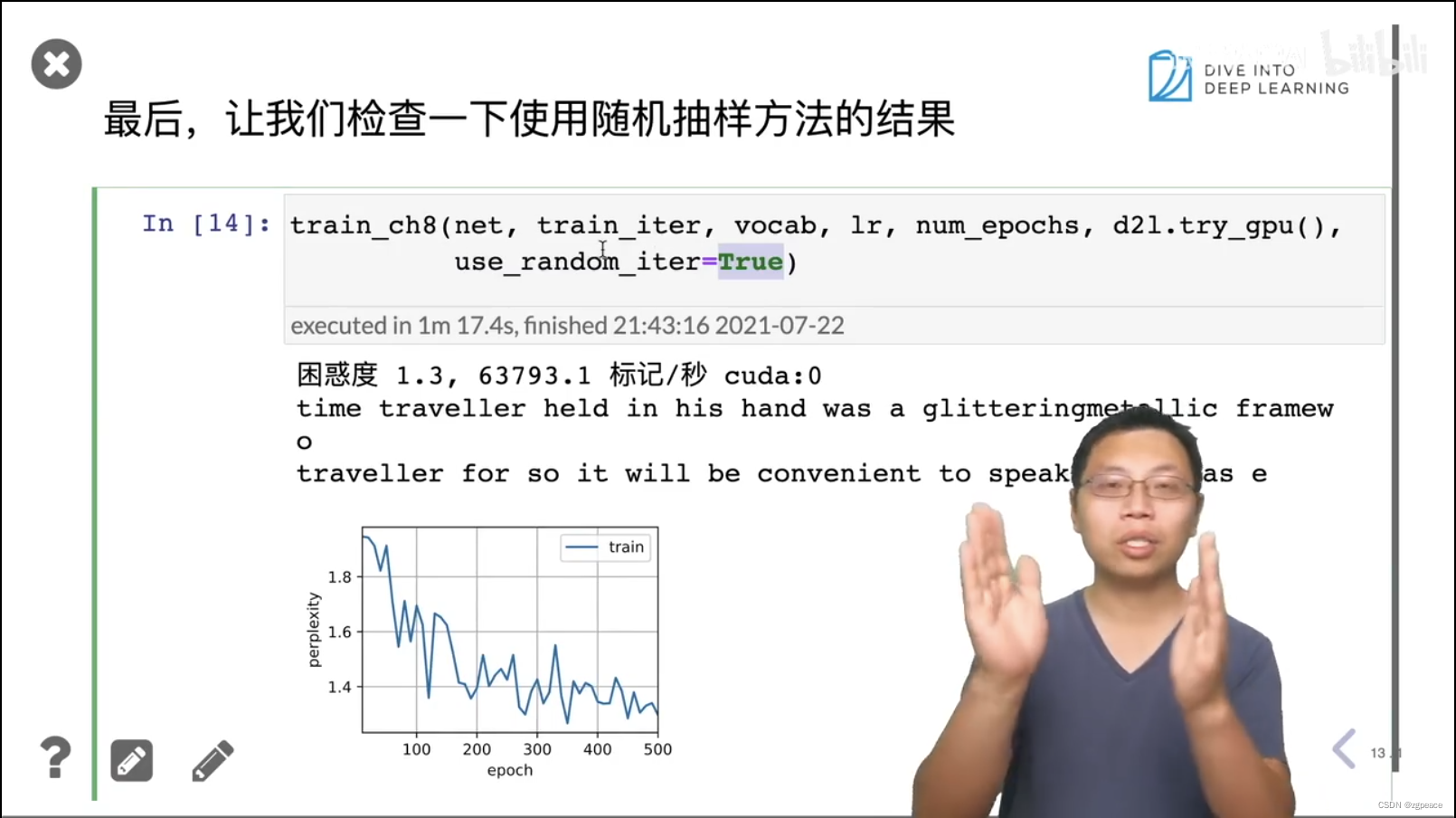# 3. 简洁实现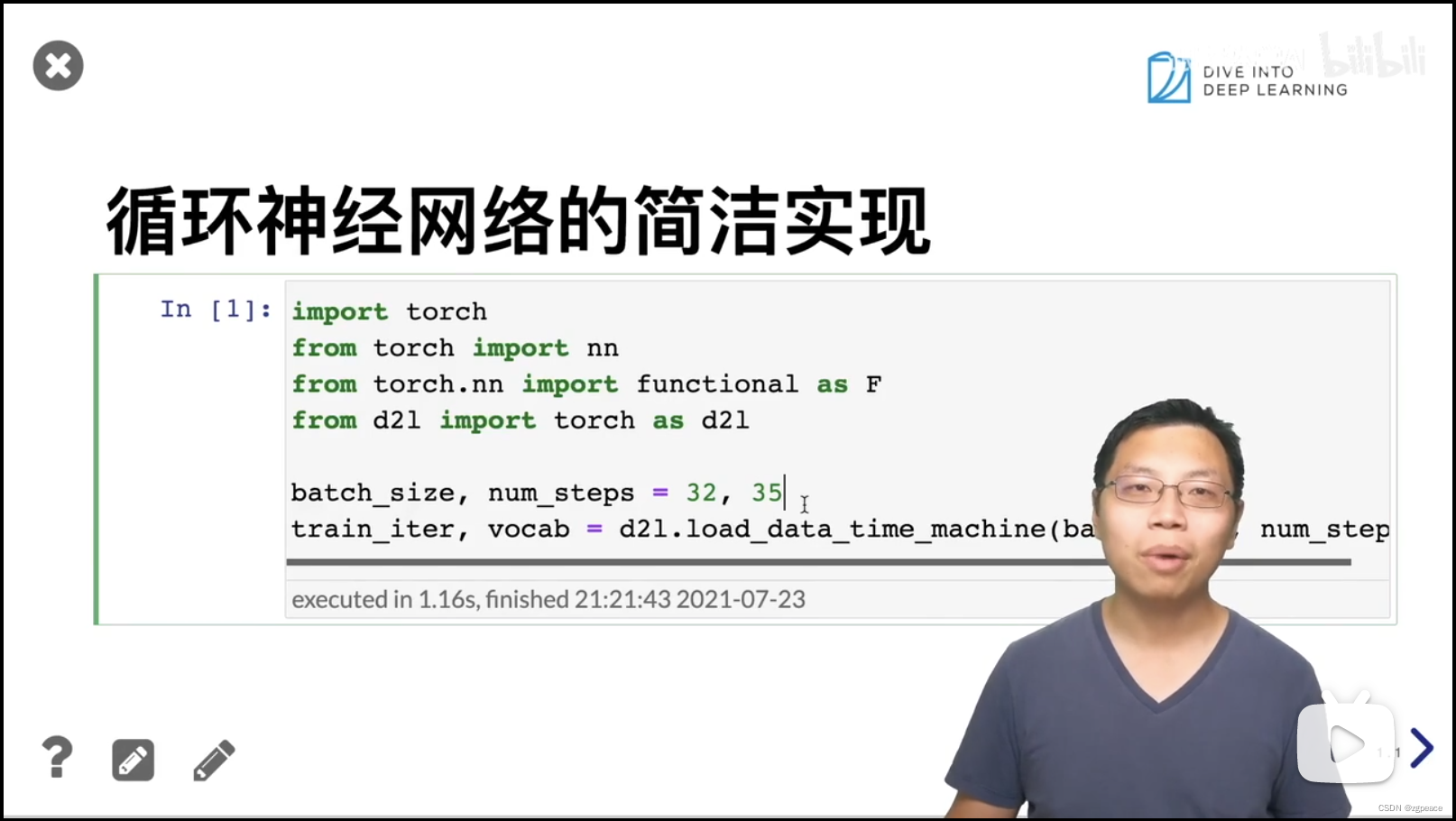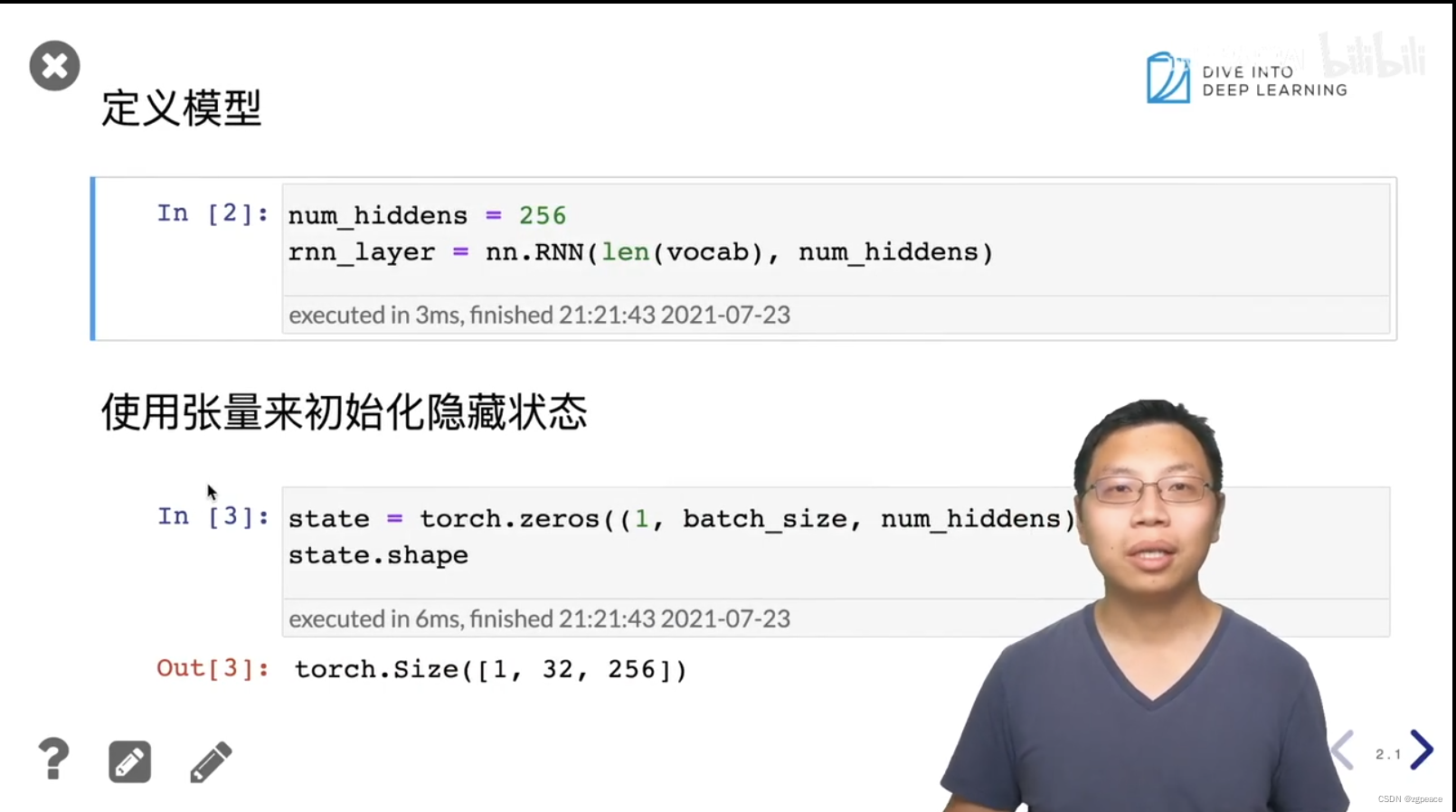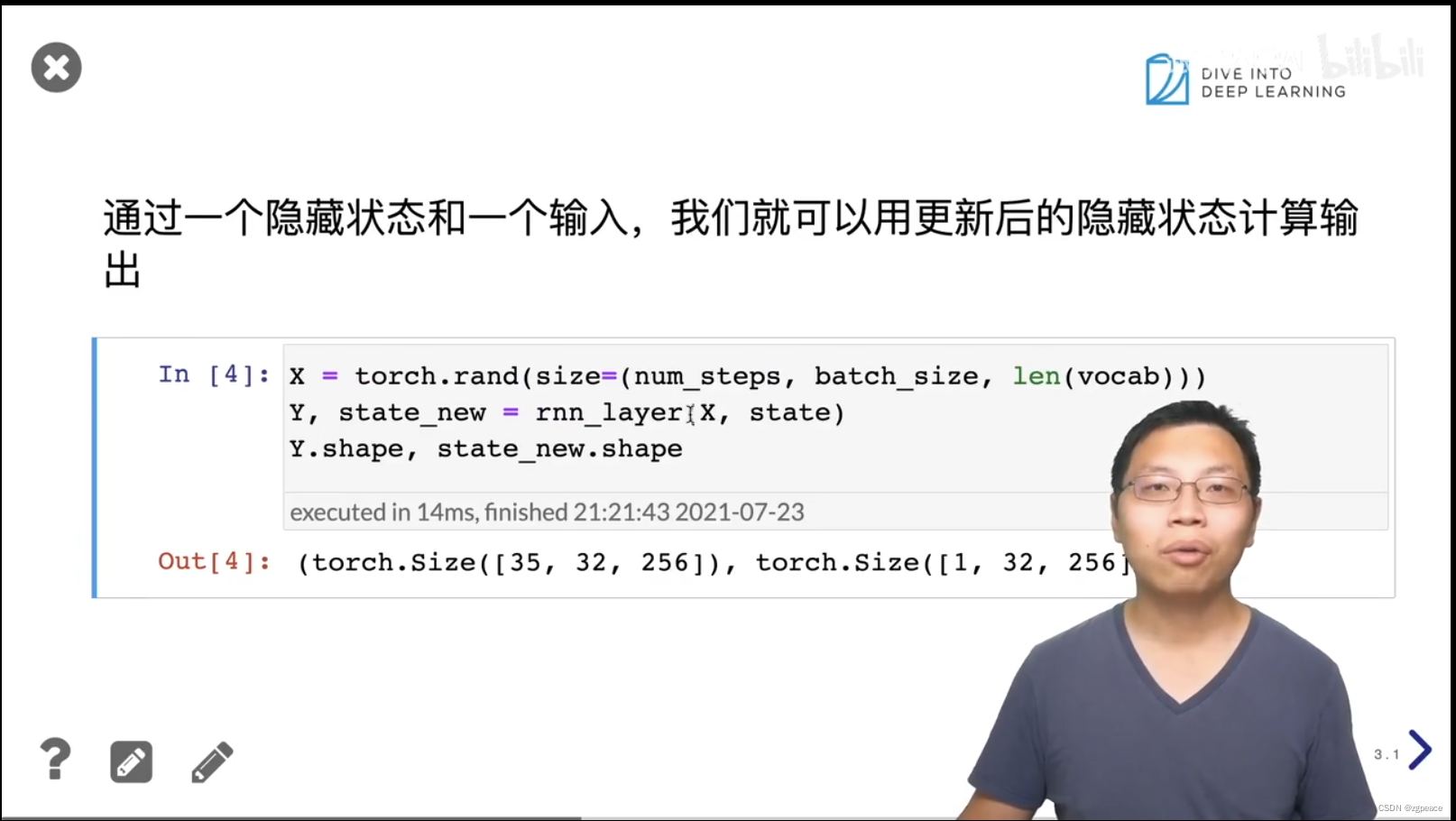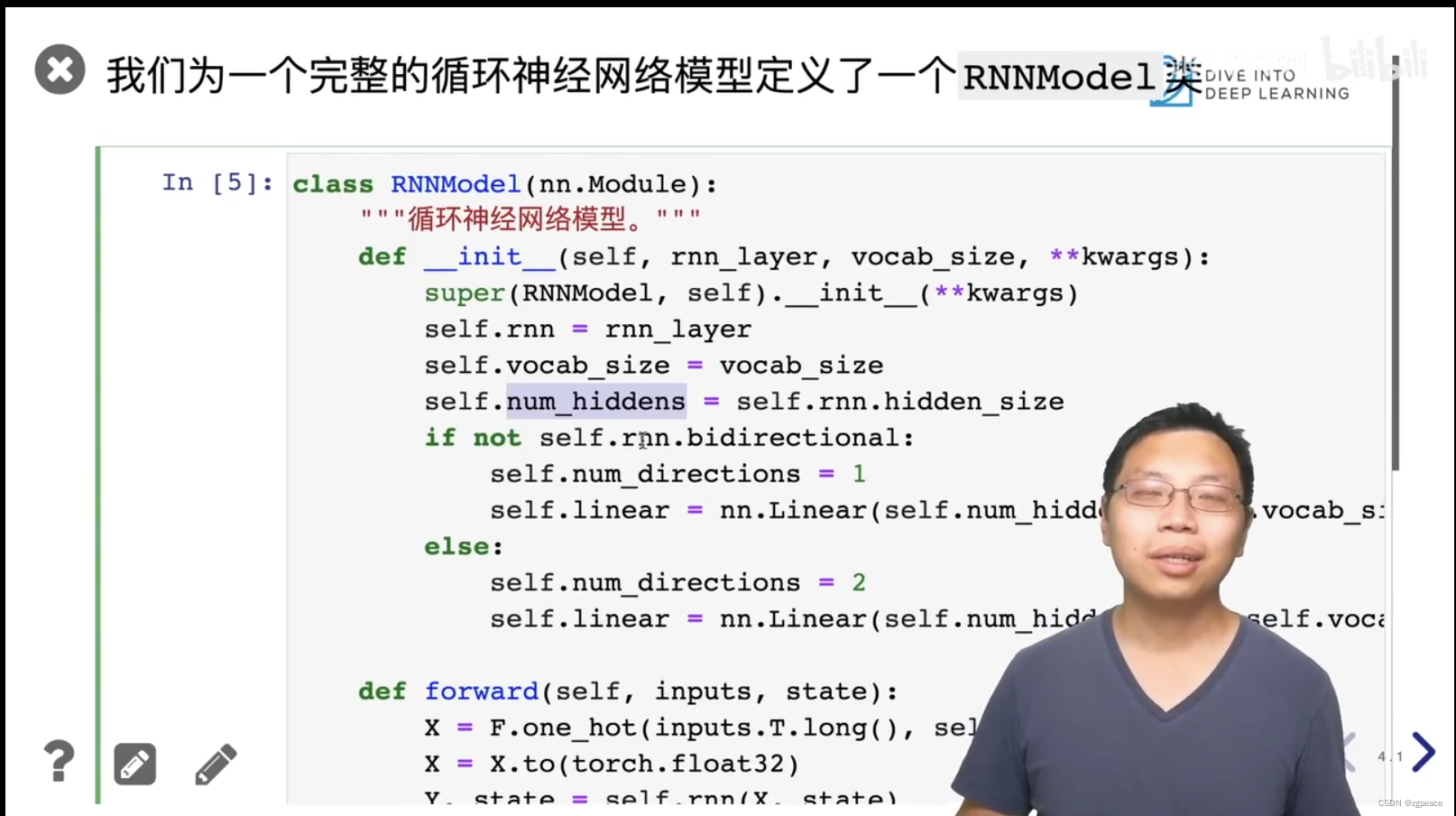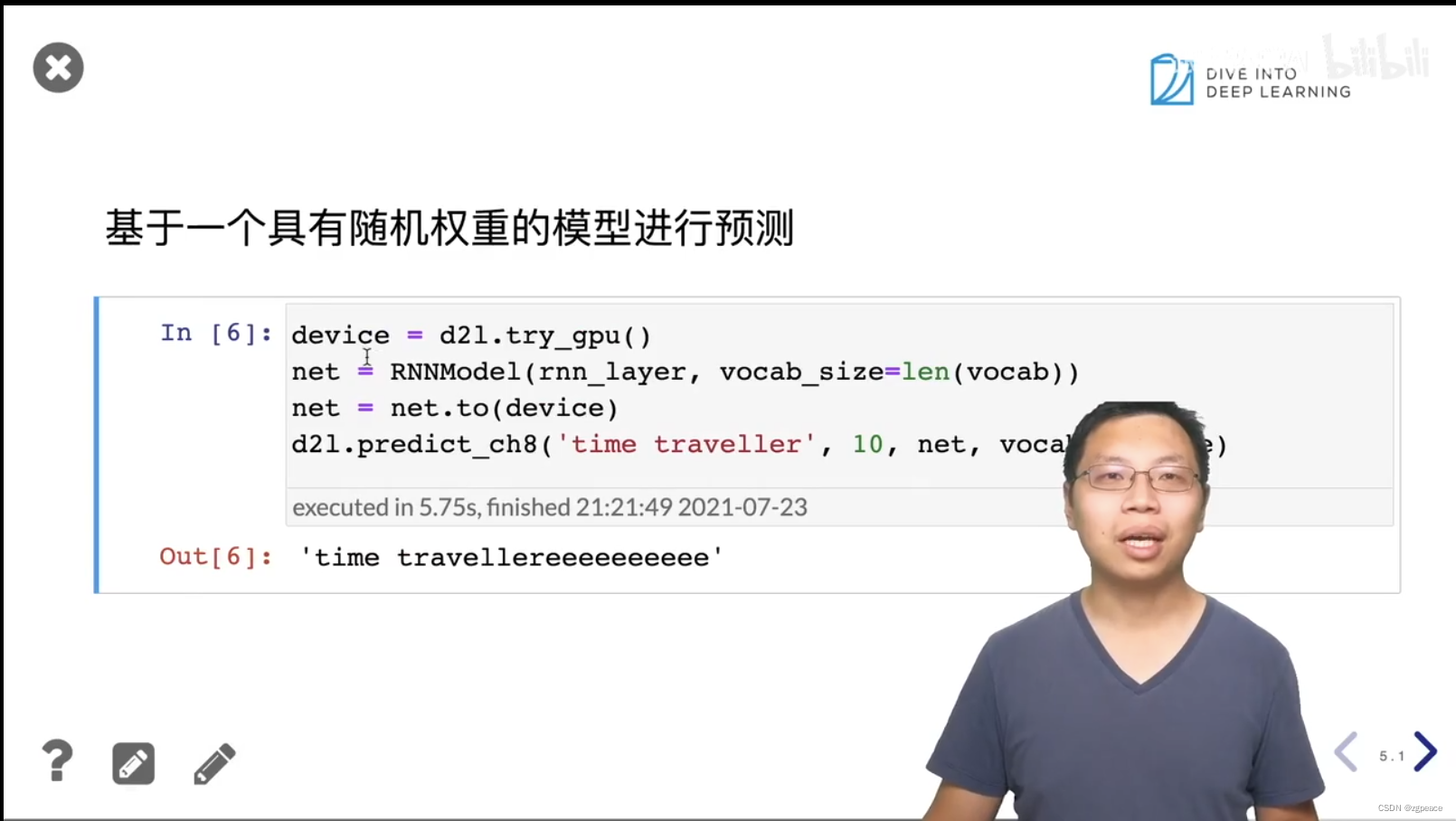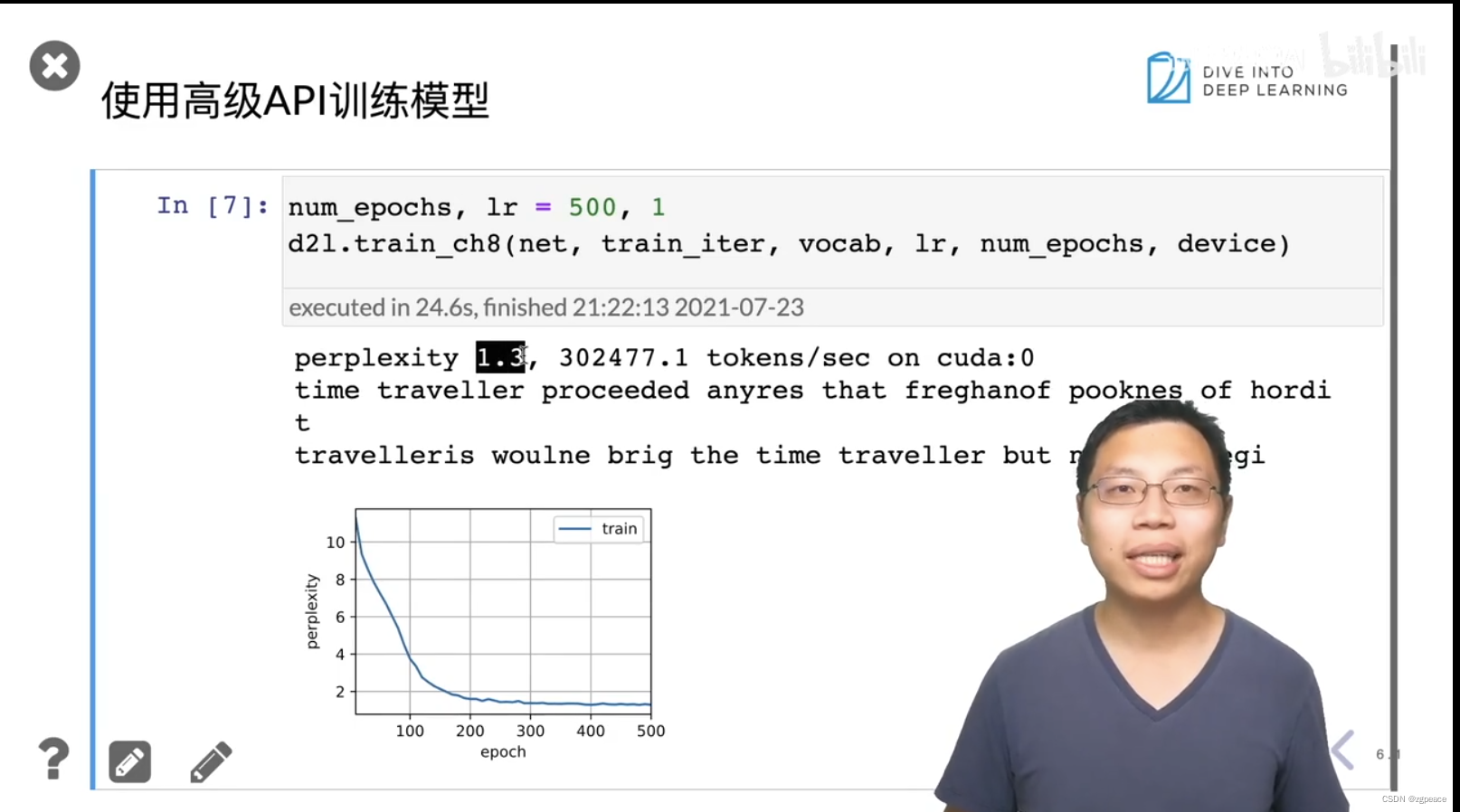# 4. Q&A

1. GPT3, BERT 都是基于Transformer模型的改进，目前也是最火的。
1. voice和image融合算法，用多模态模型。比如自动驾驶领域的运用。
1. RNN批量大小乘以时间长度t，相当于做t次分类。
1. 无人车 所有的模型都可以在车里跑，前景会比较好，车厂的钱比较多，投钱也会多。车内的视觉娱乐任务，车外的道路的视觉任务。

# 参考

https://www.bilibili.com/video/BV1D64y1z7CA/

展开全文• 目录循环神经网络RNN1.公式推导2.代码实现 循环神经网络RNN 1.公式推导 对于该循环神经网络，以中间的RNN单元为例，推导前向传播： 对于Layer-1： zh=wix+whah−1z^h = w^ix+w^ha^{h-1}zh=wix+whah−1 ah=σ(zh)a^h...机器学习 python
• 一、写在前面 这部分内容应该算是近几年发展中最基础的部分了，但是发现自己忘得差不多了...二、循环（递归）神经网络RNN 1、神经网络 在开始RNN之前我们先简单的回顾一下神经网络，下图就是一个简单的神经网络的示...深度学习 人工智能
•人工智能 深度学习
• 循环神经网络RNN）是一种专门处理序列的神经网络。它们通常用于自然语言处理(NLP)任务，因为它们在处理文本方面非常有效。在本文中，我们将探索什么是RNN，了解它们是如何工作的，并使用Python从头构建一个真正的...人工智能
•自然语言处理 nlp 人工智能 深度学习
• 循环神经网络RNN - Recurrent Neural Network Ps 循环神经网络Recurrent Neural Network 和递归神经网络Recursive Neural Network虽然缩写都是RNN ，但实际上是不一样的。 RNN(Recurrent Neural Network)是什么？ ...
• 主要介绍了循环神经网络RNN和长短时记忆网络LSTM，并对其中的原理和网络结构进行了详细的推导和解释。
• 本文将介绍经典的网络之循环神经网络RNN, Recurrent Neural Networks），这一网络也是时序数据的首选网络。当涉及某些顺序机器学习任务时，RNN可以达到很高的精度，没有其他算法可以与之一较高下。这是由于传统的......